Gromacs  2016.4
SIMD intrinsics interface (simd)Collaboration diagram for SIMD intrinsics interface (simd):

## Description

Provides an architecture-independent way of doing SIMD coding.

Overview of the SIMD implementation is provided in Single-instruction Multiple-data (SIMD) coding. The details are documented in gromacs/simd/simd.h and the reference implementation impl_reference.h.

## Namespaces

gmx
Generic GROMACS namespace.

## SIMD implementation capability definitions

#define GMX_SIMD   1
1 if any SIMD support is present, otherwise 0.

#define GMX_SIMD_HAVE_FLOAT   1
1 when SIMD float support is present, otherwise 0 More...

#define GMX_SIMD_HAVE_DOUBLE   1
1 if SIMD double support is present, otherwise 0

1 if the SIMD implementation supports unaligned loads, otherwise 0

#define GMX_SIMD_HAVE_STOREU   1
1 if the SIMD implementation supports unaligned stores, otherwise 0

#define GMX_SIMD_HAVE_FMA   0
1 if the SIMD implementation has fused-multiply add hardware More...

#define GMX_SIMD_HAVE_LOGICAL   1
1 if SIMD impl has logical operations on floating-point data, otherwise 0

#define GMX_SIMD_HAVE_FINT32_EXTRACT   1
Support for extracting integers from gmx::SimdFInt32 (1/0 for present/absent)

#define GMX_SIMD_HAVE_FINT32_LOGICAL   1
1 if SIMD logical ops are supported for gmx::SimdFInt32, otherwise 0

#define GMX_SIMD_HAVE_FINT32_ARITHMETICS   1
1 if SIMD arithmetic ops are supported for gmx::SimdFInt32, otherwise 0

#define GMX_SIMD_HAVE_DINT32_EXTRACT   1
Support for extracting integer from gmx::SimdDInt32 (1/0 for present/absent)

#define GMX_SIMD_HAVE_DINT32_LOGICAL   1
1 if logical operations are supported for gmx::SimdDInt32, otherwise 0

#define GMX_SIMD_HAVE_DINT32_ARITHMETICS   1
1 if SIMD arithmetic ops are supported for gmx::SimdDInt32, otherwise 0

#define GMX_SIMD_HAVE_NATIVE_COPYSIGN_FLOAT   0
1 if implementation provides single precision copysign() More...

#define GMX_SIMD_HAVE_NATIVE_RSQRT_ITER_FLOAT   0
1 if implementation provides single precision 1/sqrt(x) N-R iterations faster than simd_math.h More...

#define GMX_SIMD_HAVE_NATIVE_RCP_ITER_FLOAT   0
1 if implementation provides single precision 1/x N-R iterations faster than simd_math.h More...

#define GMX_SIMD_HAVE_NATIVE_LOG_FLOAT   0
1 if implementation provides single precision log() faster than simd_math.h More...

#define GMX_SIMD_HAVE_NATIVE_EXP2_FLOAT   0
1 if implementation provides single precision exp2() faster than simd_math.h More...

#define GMX_SIMD_HAVE_NATIVE_EXP_FLOAT   0
1 if implementation provides single precision exp() faster than simd_math.h More...

#define GMX_SIMD_HAVE_NATIVE_COPYSIGN_DOUBLE   0
1 if implementation provides double precision copysign() More...

#define GMX_SIMD_HAVE_NATIVE_RSQRT_ITER_DOUBLE   0
1 if implementation provides double precision 1/sqrt(x) N-R iterations faster than simd_math.h More...

#define GMX_SIMD_HAVE_NATIVE_RCP_ITER_DOUBLE   0
1 if implementation provides double precision 1/x N-R iterations faster than simd_math.h More...

#define GMX_SIMD_HAVE_NATIVE_LOG_DOUBLE   0
1 if implementation provides double precision log() faster than simd_math.h More...

#define GMX_SIMD_HAVE_NATIVE_EXP2_DOUBLE   0
1 if implementation provides double precision exp2() faster than simd_math.h More...

#define GMX_SIMD_HAVE_NATIVE_EXP_DOUBLE   0
1 if implementation provides double precision exp() faster than simd_math.h More...

1 if gmx::gatherLoadUBySimdIntTranspose is present, otherwise 0

1 if gmx::gatherLoadUBySimdIntTranspose is present, otherwise 0

#define GMX_SIMD_HAVE_HSIMD_UTIL_FLOAT   1
1 if float half-register load/store/reduce utils present, otherwise 0

#define GMX_SIMD_HAVE_HSIMD_UTIL_DOUBLE   1
1 if double half-register load/store/reduce utils present, otherwise 0

#define GMX_SIMD_FLOAT_WIDTH   4
Width of the gmx::SimdFloat datatype.

#define GMX_SIMD_DOUBLE_WIDTH   4
Width of the gmx::SimdDouble datatype.

#define GMX_SIMD4_HAVE_FLOAT   1
1 if implementation provides gmx::Simd4Float, otherwise 0.

#define GMX_SIMD4_HAVE_DOUBLE   1
1 if the implementation provides gmx::Simd4Double, otherwise 0.

#define GMX_SIMD_FINT32_WIDTH   GMX_SIMD_FLOAT_WIDTH
Width of the gmx::SimdFInt32 datatype.

#define GMX_SIMD_DINT32_WIDTH   GMX_SIMD_DOUBLE_WIDTH
Width of the gmx::SimdDInt32 datatype.

#define GMX_SIMD4_WIDTH   4
The SIMD4 type is always four units wide, but this makes code more explicit.

#define GMX_SIMD_RSQRT_BITS   23
Accuracy of SIMD 1/sqrt(x) lookup. Used to determine number of iterations.

#define GMX_SIMD_RCP_BITS   23
Accuracy of SIMD 1/x lookup. Used to determine number of iterations.

## Constant width-4 double precision SIMD types and instructions

static Simd4Double gmx_simdcall gmx::load4 (const double *m)
Load 4 double values from aligned memory into SIMD4 variable. More...

static void gmx_simdcall gmx::store4 (double *m, Simd4Double a)
Store the contents of SIMD4 double to aligned memory m. More...

static Simd4Double gmx_simdcall gmx::load4U (const double *m)
Load SIMD4 double from unaligned memory. More...

static void gmx_simdcall gmx::store4U (double *m, Simd4Double a)
Store SIMD4 double to unaligned memory. More...

static Simd4Double gmx_simdcall gmx::simd4SetZeroD ()
Set all SIMD4 double elements to 0. More...

static Simd4Double gmx_simdcall gmx::operator& (Simd4Double a, Simd4Double b)
Bitwise and for two SIMD4 double variables. More...

static Simd4Double gmx_simdcall gmx::andNot (Simd4Double a, Simd4Double b)
Bitwise andnot for two SIMD4 double variables. c=(~a) & b. More...

static Simd4Double gmx_simdcall gmx::operator| (Simd4Double a, Simd4Double b)
Bitwise or for two SIMD4 doubles. More...

static Simd4Double gmx_simdcall gmx::operator^ (Simd4Double a, Simd4Double b)
Bitwise xor for two SIMD4 double variables. More...

static Simd4Double gmx_simdcall gmx::operator+ (Simd4Double a, Simd4Double b)
Add two double SIMD4 variables. More...

static Simd4Double gmx_simdcall gmx::operator- (Simd4Double a, Simd4Double b)
Subtract two SIMD4 variables. More...

static Simd4Double gmx_simdcall gmx::operator- (Simd4Double a)
SIMD4 floating-point negate. More...

static Simd4Double gmx_simdcall gmx::operator* (Simd4Double a, Simd4Double b)
Multiply two SIMD4 variables. More...

static Simd4Double gmx_simdcall gmx::fma (Simd4Double a, Simd4Double b, Simd4Double c)
SIMD4 Fused-multiply-add. Result is a*b+c. More...

static Simd4Double gmx_simdcall gmx::fms (Simd4Double a, Simd4Double b, Simd4Double c)
SIMD4 Fused-multiply-subtract. Result is a*b-c. More...

static Simd4Double gmx_simdcall gmx::fnma (Simd4Double a, Simd4Double b, Simd4Double c)
SIMD4 Fused-negated-multiply-add. Result is -a*b+c. More...

static Simd4Double gmx_simdcall gmx::fnms (Simd4Double a, Simd4Double b, Simd4Double c)
SIMD4 Fused-negated-multiply-subtract. Result is -a*b-c. More...

static Simd4Double gmx_simdcall gmx::rsqrt (Simd4Double x)
SIMD4 1.0/sqrt(x) lookup. More...

static Simd4Double gmx_simdcall gmx::abs (Simd4Double a)
SIMD4 Floating-point abs(). More...

static Simd4Double gmx_simdcall gmx::max (Simd4Double a, Simd4Double b)
Set each SIMD4 element to the largest from two variables. More...

static Simd4Double gmx_simdcall gmx::min (Simd4Double a, Simd4Double b)
Set each SIMD4 element to the largest from two variables. More...

static Simd4Double gmx_simdcall gmx::round (Simd4Double a)
SIMD4 Round to nearest integer value (in floating-point format). More...

static Simd4Double gmx_simdcall gmx::trunc (Simd4Double a)
Truncate SIMD4, i.e. round towards zero - common hardware instruction. More...

static double gmx_simdcall gmx::dotProduct (Simd4Double a, Simd4Double b)
Return dot product of two double precision SIMD4 variables. More...

static void gmx_simdcall gmx::transpose (Simd4Double *v0, Simd4Double *v1, Simd4Double *v2, Simd4Double *v3)
SIMD4 double transpose. More...

static Simd4DBool gmx_simdcall gmx::operator== (Simd4Double a, Simd4Double b)
a==b for SIMD4 double More...

static Simd4DBool gmx_simdcall gmx::operator!= (Simd4Double a, Simd4Double b)
a!=b for SIMD4 double More...

static Simd4DBool gmx_simdcall gmx::operator< (Simd4Double a, Simd4Double b)
a<b for SIMD4 double More...

static Simd4DBool gmx_simdcall gmx::operator<= (Simd4Double a, Simd4Double b)
a<=b for SIMD4 double. More...

static Simd4DBool gmx_simdcall gmx::operator&& (Simd4DBool a, Simd4DBool b)
Logical and on single precision SIMD4 booleans. More...

static Simd4DBool gmx_simdcall gmx::operator|| (Simd4DBool a, Simd4DBool b)
Logical or on single precision SIMD4 booleans. More...

static bool gmx_simdcall gmx::anyTrue (Simd4DBool a)
Returns non-zero if any of the boolean in SIMD4 a is True, otherwise 0. More...

static Simd4Double gmx_simdcall gmx::selectByMask (Simd4Double a, Simd4DBool mask)
Select from single precision SIMD4 variable where boolean is true. More...

static Simd4Double gmx_simdcall gmx::selectByNotMask (Simd4Double a, Simd4DBool mask)
Select from single precision SIMD4 variable where boolean is false. More...

static Simd4Double gmx_simdcall gmx::blend (Simd4Double a, Simd4Double b, Simd4DBool sel)
Vector-blend SIMD4 selection. More...

static double gmx_simdcall gmx::reduce (Simd4Double a)
Return sum of all elements in SIMD4 double variable. More...

## Constant width-4 single precision SIMD types and instructions

static Simd4Float gmx_simdcall gmx::load4 (const float *m)
Load 4 float values from aligned memory into SIMD4 variable. More...

static void gmx_simdcall gmx::store4 (float *m, Simd4Float a)
Store the contents of SIMD4 float to aligned memory m. More...

static Simd4Float gmx_simdcall gmx::load4U (const float *m)
Load SIMD4 float from unaligned memory. More...

static void gmx_simdcall gmx::store4U (float *m, Simd4Float a)
Store SIMD4 float to unaligned memory. More...

static Simd4Float gmx_simdcall gmx::simd4SetZeroF ()
Set all SIMD4 float elements to 0. More...

static Simd4Float gmx_simdcall gmx::operator& (Simd4Float a, Simd4Float b)
Bitwise and for two SIMD4 float variables. More...

static Simd4Float gmx_simdcall gmx::andNot (Simd4Float a, Simd4Float b)
Bitwise andnot for two SIMD4 float variables. c=(~a) & b. More...

static Simd4Float gmx_simdcall gmx::operator| (Simd4Float a, Simd4Float b)
Bitwise or for two SIMD4 floats. More...

static Simd4Float gmx_simdcall gmx::operator^ (Simd4Float a, Simd4Float b)
Bitwise xor for two SIMD4 float variables. More...

static Simd4Float gmx_simdcall gmx::operator+ (Simd4Float a, Simd4Float b)
Add two float SIMD4 variables. More...

static Simd4Float gmx_simdcall gmx::operator- (Simd4Float a, Simd4Float b)
Subtract two SIMD4 variables. More...

static Simd4Float gmx_simdcall gmx::operator- (Simd4Float a)
SIMD4 floating-point negate. More...

static Simd4Float gmx_simdcall gmx::operator* (Simd4Float a, Simd4Float b)
Multiply two SIMD4 variables. More...

static Simd4Float gmx_simdcall gmx::fma (Simd4Float a, Simd4Float b, Simd4Float c)
SIMD4 Fused-multiply-add. Result is a*b+c. More...

static Simd4Float gmx_simdcall gmx::fms (Simd4Float a, Simd4Float b, Simd4Float c)
SIMD4 Fused-multiply-subtract. Result is a*b-c. More...

static Simd4Float gmx_simdcall gmx::fnma (Simd4Float a, Simd4Float b, Simd4Float c)
SIMD4 Fused-negated-multiply-add. Result is -a*b+c. More...

static Simd4Float gmx_simdcall gmx::fnms (Simd4Float a, Simd4Float b, Simd4Float c)
SIMD4 Fused-negated-multiply-subtract. Result is -a*b-c. More...

static Simd4Float gmx_simdcall gmx::rsqrt (Simd4Float x)
SIMD4 1.0/sqrt(x) lookup. More...

static Simd4Float gmx_simdcall gmx::abs (Simd4Float a)
SIMD4 Floating-point fabs(). More...

static Simd4Float gmx_simdcall gmx::max (Simd4Float a, Simd4Float b)
Set each SIMD4 element to the largest from two variables. More...

static Simd4Float gmx_simdcall gmx::min (Simd4Float a, Simd4Float b)
Set each SIMD4 element to the largest from two variables. More...

static Simd4Float gmx_simdcall gmx::round (Simd4Float a)
SIMD4 Round to nearest integer value (in floating-point format). More...

static Simd4Float gmx_simdcall gmx::trunc (Simd4Float a)
Truncate SIMD4, i.e. round towards zero - common hardware instruction. More...

static float gmx_simdcall gmx::dotProduct (Simd4Float a, Simd4Float b)
Return dot product of two single precision SIMD4 variables. More...

static void gmx_simdcall gmx::transpose (Simd4Float *v0, Simd4Float *v1, Simd4Float *v2, Simd4Float *v3)
SIMD4 float transpose. More...

static Simd4FBool gmx_simdcall gmx::operator== (Simd4Float a, Simd4Float b)
a==b for SIMD4 float More...

static Simd4FBool gmx_simdcall gmx::operator!= (Simd4Float a, Simd4Float b)
a!=b for SIMD4 float More...

static Simd4FBool gmx_simdcall gmx::operator< (Simd4Float a, Simd4Float b)
a<b for SIMD4 float More...

static Simd4FBool gmx_simdcall gmx::operator<= (Simd4Float a, Simd4Float b)
a<=b for SIMD4 float. More...

static Simd4FBool gmx_simdcall gmx::operator&& (Simd4FBool a, Simd4FBool b)
Logical and on single precision SIMD4 booleans. More...

static Simd4FBool gmx_simdcall gmx::operator|| (Simd4FBool a, Simd4FBool b)
Logical or on single precision SIMD4 booleans. More...

static bool gmx_simdcall gmx::anyTrue (Simd4FBool a)
Returns non-zero if any of the boolean in SIMD4 a is True, otherwise 0. More...

static Simd4Float gmx_simdcall gmx::selectByMask (Simd4Float a, Simd4FBool mask)
Select from single precision SIMD4 variable where boolean is true. More...

static Simd4Float gmx_simdcall gmx::selectByNotMask (Simd4Float a, Simd4FBool mask)
Select from single precision SIMD4 variable where boolean is false. More...

static Simd4Float gmx_simdcall gmx::blend (Simd4Float a, Simd4Float b, Simd4FBool sel)
Vector-blend SIMD4 selection. More...

static float gmx_simdcall gmx::reduce (Simd4Float a)
Return sum of all elements in SIMD4 float variable. More...

## SIMD predefined macros to describe high-level capabilities

These macros are used to describe the features available in default Gromacs real precision. They are set from the lower-level implementation files that have macros describing single and double precision individually, as well as the implementation details.

#define GMX_SIMD_HAVE_REAL   GMX_SIMD_HAVE_FLOAT
1 if SimdReal is available, otherwise 0. More...

#define GMX_SIMD_REAL_WIDTH   GMX_SIMD_FLOAT_WIDTH
Width of SimdReal. More...

#define GMX_SIMD_HAVE_INT32_EXTRACT   GMX_SIMD_HAVE_FINT32_EXTRACT
1 if support is available for extracting elements from SimdInt32, otherwise 0 More...

#define GMX_SIMD_HAVE_INT32_LOGICAL   GMX_SIMD_HAVE_FINT32_LOGICAL
1 if logical ops are supported on SimdInt32, otherwise 0. More...

#define GMX_SIMD_HAVE_INT32_ARITHMETICS   GMX_SIMD_HAVE_FINT32_ARITHMETICS
1 if arithmetic ops are supported on SimdInt32, otherwise 0. More...

1 if gmx::simdGatherLoadUBySimdIntTranspose is present, otherwise 0 More...

#define GMX_SIMD_HAVE_HSIMD_UTIL_REAL   GMX_SIMD_HAVE_HSIMD_UTIL_FLOAT
1 if real half-register load/store/reduce utils present, otherwise 0 More...

#define GMX_SIMD4_HAVE_REAL   GMX_SIMD4_HAVE_FLOAT
1 if Simd4Real is available, otherwise 0. More...

## Single precision SIMD math functions

Note
In most cases you should use the real-precision functions instead.
static SimdFloat gmx_simdcall gmx::copysign (SimdFloat x, SimdFloat y)
Composes floating point value with the magnitude of x and the sign of y. More...

static SimdFloat gmx_simdcall gmx::rsqrtIter (SimdFloat lu, SimdFloat x)
Perform one Newton-Raphson iteration to improve 1/sqrt(x) for SIMD float. More...

static SimdFloat gmx_simdcall gmx::invsqrt (SimdFloat x)
Calculate 1/sqrt(x) for SIMD float. More...

static void gmx_simdcall gmx::invsqrtPair (SimdFloat x0, SimdFloat x1, SimdFloat *out0, SimdFloat *out1)
Calculate 1/sqrt(x) for two SIMD floats. More...

static SimdFloat gmx_simdcall gmx::rcpIter (SimdFloat lu, SimdFloat x)
Perform one Newton-Raphson iteration to improve 1/x for SIMD float. More...

static SimdFloat gmx_simdcall gmx::inv (SimdFloat x)
Calculate 1/x for SIMD float. More...

static SimdFloat gmx_simdcall gmx::operator/ (SimdFloat nom, SimdFloat denom)
Division for SIMD floats. More...

static SimdFloat gmx::maskzInvsqrt (SimdFloat x, SimdFBool m)
Calculate 1/sqrt(x) for masked entries of SIMD float. More...

static SimdFloat gmx_simdcall gmx::maskzInv (SimdFloat x, SimdFBool m)
Calculate 1/x for SIMD float, masked version. More...

static SimdFloat gmx_simdcall gmx::sqrt (SimdFloat x)
Calculate sqrt(x) correctly for SIMD floats, including argument 0.0. More...

static SimdFloat gmx_simdcall gmx::log (SimdFloat x)
SIMD float log(x). This is the natural logarithm. More...

static SimdFloat gmx_simdcall gmx::exp2 (SimdFloat x)
SIMD float 2^x. More...

static SimdFloat gmx_simdcall gmx::exp (SimdFloat x)
SIMD float exp(x). More...

static SimdFloat gmx_simdcall gmx::erf (SimdFloat x)
SIMD float erf(x). More...

static SimdFloat gmx_simdcall gmx::erfc (SimdFloat x)
SIMD float erfc(x). More...

static void gmx_simdcall gmx::sincos (SimdFloat x, SimdFloat *sinval, SimdFloat *cosval)
SIMD float sin & cos. More...

static SimdFloat gmx_simdcall gmx::sin (SimdFloat x)
SIMD float sin(x). More...

static SimdFloat gmx_simdcall gmx::cos (SimdFloat x)
SIMD float cos(x). More...

static SimdFloat gmx_simdcall gmx::tan (SimdFloat x)
SIMD float tan(x). More...

static SimdFloat gmx_simdcall gmx::asin (SimdFloat x)
SIMD float asin(x). More...

static SimdFloat gmx_simdcall gmx::acos (SimdFloat x)
SIMD float acos(x). More...

static SimdFloat gmx_simdcall gmx::atan (SimdFloat x)
SIMD float asin(x). More...

static SimdFloat gmx_simdcall gmx::atan2 (SimdFloat y, SimdFloat x)
SIMD float atan2(y,x). More...

static SimdFloat gmx_simdcall gmx::pmeForceCorrection (SimdFloat z2)
Calculate the force correction due to PME analytically in SIMD float. More...

static SimdFloat gmx_simdcall gmx::pmePotentialCorrection (SimdFloat z2)
Calculate the potential correction due to PME analytically in SIMD float. More...

## Double precision SIMD math functions

Note
In most cases you should use the real-precision functions instead.
static SimdDouble gmx_simdcall gmx::copysign (SimdDouble x, SimdDouble y)
Composes floating point value with the magnitude of x and the sign of y. More...

static SimdDouble gmx_simdcall gmx::rsqrtIter (SimdDouble lu, SimdDouble x)
Perform one Newton-Raphson iteration to improve 1/sqrt(x) for SIMD double. More...

static SimdDouble gmx_simdcall gmx::invsqrt (SimdDouble x)
Calculate 1/sqrt(x) for SIMD double. More...

static void gmx_simdcall gmx::invsqrtPair (SimdDouble x0, SimdDouble x1, SimdDouble *out0, SimdDouble *out1)
Calculate 1/sqrt(x) for two SIMD doubles. More...

static SimdDouble gmx_simdcall gmx::rcpIter (SimdDouble lu, SimdDouble x)
Perform one Newton-Raphson iteration to improve 1/x for SIMD double. More...

static SimdDouble gmx_simdcall gmx::inv (SimdDouble x)
Calculate 1/x for SIMD double. More...

static SimdDouble gmx_simdcall gmx::operator/ (SimdDouble nom, SimdDouble denom)
Division for SIMD doubles. More...

static SimdDouble gmx::maskzInvsqrt (SimdDouble x, SimdDBool m)
Calculate 1/sqrt(x) for masked entries of SIMD double. More...

static SimdDouble gmx_simdcall gmx::maskzInv (SimdDouble x, SimdDBool m)
Calculate 1/x for SIMD double, masked version. More...

static SimdDouble gmx_simdcall gmx::sqrt (SimdDouble x)
Calculate sqrt(x) correctly for SIMD doubles, including argument 0.0. More...

static SimdDouble gmx_simdcall gmx::log (SimdDouble x)
SIMD double log(x). This is the natural logarithm. More...

static SimdDouble gmx_simdcall gmx::exp2 (SimdDouble x)
SIMD double 2^x. More...

static SimdDouble gmx_simdcall gmx::exp (SimdDouble x)
SIMD double exp(x). More...

static SimdDouble gmx_simdcall gmx::erf (SimdDouble x)
SIMD double erf(x). More...

static SimdDouble gmx_simdcall gmx::erfc (SimdDouble x)
SIMD double erfc(x). More...

static void gmx_simdcall gmx::sincos (SimdDouble x, SimdDouble *sinval, SimdDouble *cosval)
SIMD double sin & cos. More...

static SimdDouble gmx_simdcall gmx::sin (SimdDouble x)
SIMD double sin(x). More...

static SimdDouble gmx_simdcall gmx::cos (SimdDouble x)
SIMD double cos(x). More...

static SimdDouble gmx_simdcall gmx::tan (SimdDouble x)
SIMD double tan(x). More...

static SimdDouble gmx_simdcall gmx::asin (SimdDouble x)
SIMD double asin(x). More...

static SimdDouble gmx_simdcall gmx::acos (SimdDouble x)
SIMD double acos(x). More...

static SimdDouble gmx_simdcall gmx::atan (SimdDouble x)
SIMD double asin(x). More...

static SimdDouble gmx_simdcall gmx::atan2 (SimdDouble y, SimdDouble x)
SIMD double atan2(y,x). More...

static SimdDouble gmx_simdcall gmx::pmeForceCorrection (SimdDouble z2)
Calculate the force correction due to PME analytically in SIMD double. More...

static SimdDouble gmx_simdcall gmx::pmePotentialCorrection (SimdDouble z2)
Calculate the potential correction due to PME analytically in SIMD double. More...

## SIMD math functions for double prec. data, single prec. accuracy

Note
In some cases we do not need full double accuracy of individual SIMD math functions, although the data is stored in double precision SIMD registers. This might be the case for special algorithms, or if the architecture does not support single precision. Since the full double precision evaluation of math functions typically require much more expensive polynomial approximations these functions implement the algorithms used in the single precision SIMD math functions, but they operate on double precision SIMD variables.
static SimdDouble gmx_simdcall gmx::invsqrtSingleAccuracy (SimdDouble x)
Calculate 1/sqrt(x) for SIMD double, but in single accuracy. More...

static SimdDouble gmx::maskzInvsqrtSingleAccuracy (SimdDouble x, SimdDBool m)
1/sqrt(x) for masked-in entries of SIMD double, but in single accuracy. More...

static void gmx_simdcall gmx::invsqrtPairSingleAccuracy (SimdDouble x0, SimdDouble x1, SimdDouble *out0, SimdDouble *out1)
Calculate 1/sqrt(x) for two SIMD doubles, but single accuracy. More...

static SimdDouble gmx_simdcall gmx::invSingleAccuracy (SimdDouble x)
Calculate 1/x for SIMD double, but in single accuracy. More...

static SimdDouble gmx_simdcall gmx::maskzInvSingleAccuracy (SimdDouble x, SimdDBool m)
1/x for masked entries of SIMD double, single accuracy. More...

static SimdDouble gmx_simdcall gmx::sqrtSingleAccuracy (SimdDouble x)
Calculate sqrt(x) (correct for 0.0) for SIMD double, single accuracy. More...

static SimdDouble gmx_simdcall gmx::logSingleAccuracy (SimdDouble x)
SIMD log(x). Double precision SIMD data, single accuracy. More...

static SimdDouble gmx_simdcall gmx::exp2SingleAccuracy (SimdDouble x)
SIMD 2^x. Double precision SIMD data, single accuracy. More...

static SimdDouble gmx_simdcall gmx::expSingleAccuracy (SimdDouble x)
SIMD exp(x). Double precision SIMD data, single accuracy. More...

static SimdDouble gmx_simdcall gmx::erfSingleAccuracy (SimdDouble x)
SIMD erf(x). Double precision SIMD data, single accuracy. More...

static SimdDouble gmx_simdcall gmx::erfcSingleAccuracy (SimdDouble x)
SIMD erfc(x). Double precision SIMD data, single accuracy. More...

static void gmx_simdcall gmx::sinCosSingleAccuracy (SimdDouble x, SimdDouble *sinval, SimdDouble *cosval)
SIMD sin & cos. Double precision SIMD data, single accuracy. More...

static SimdDouble gmx_simdcall gmx::sinSingleAccuracy (SimdDouble x)
SIMD sin(x). Double precision SIMD data, single accuracy. More...

static SimdDouble gmx_simdcall gmx::cosSingleAccuracy (SimdDouble x)
SIMD cos(x). Double precision SIMD data, single accuracy. More...

static SimdDouble gmx_simdcall gmx::tanSingleAccuracy (SimdDouble x)
SIMD tan(x). Double precision SIMD data, single accuracy. More...

static SimdDouble gmx_simdcall gmx::asinSingleAccuracy (SimdDouble x)
SIMD asin(x). Double precision SIMD data, single accuracy. More...

static SimdDouble gmx_simdcall gmx::acosSingleAccuracy (SimdDouble x)
SIMD acos(x). Double precision SIMD data, single accuracy. More...

static SimdDouble gmx_simdcall gmx::atanSingleAccuracy (SimdDouble x)
SIMD asin(x). Double precision SIMD data, single accuracy. More...

static SimdDouble gmx_simdcall gmx::atan2SingleAccuracy (SimdDouble y, SimdDouble x)
SIMD atan2(y,x). Double precision SIMD data, single accuracy. More...

static SimdDouble gmx_simdcall gmx::pmeForceCorrectionSingleAccuracy (SimdDouble z2)
Analytical PME force correction, double SIMD data, single accuracy. More...

static SimdDouble gmx_simdcall gmx::pmePotentialCorrectionSingleAccuracy (SimdDouble z2)
Analytical PME potential correction, double SIMD data, single accuracy. More...

## SIMD4 math functions

Note
Only a subset of the math functions are implemented for SIMD4.
static Simd4Float gmx_simdcall gmx::rsqrtIter (Simd4Float lu, Simd4Float x)
Perform one Newton-Raphson iteration to improve 1/sqrt(x) for SIMD4 float. More...

static Simd4Float gmx_simdcall gmx::invsqrt (Simd4Float x)
Calculate 1/sqrt(x) for SIMD4 float. More...

static Simd4Double gmx_simdcall gmx::rsqrtIter (Simd4Double lu, Simd4Double x)
Perform one Newton-Raphson iteration to improve 1/sqrt(x) for SIMD4 double. More...

static Simd4Double gmx_simdcall gmx::invsqrt (Simd4Double x)
Calculate 1/sqrt(x) for SIMD4 double. More...

static Simd4Double gmx_simdcall gmx::invsqrtSingleAccuracy (Simd4Double x)
Calculate 1/sqrt(x) for SIMD4 double, but in single accuracy. More...

## Classes

class  gmx::Simd4Double
SIMD4 double type. More...

class  gmx::Simd4DBool
SIMD4 variable type to use for logical comparisons on doubles. More...

class  gmx::Simd4Float
SIMD4 float type. More...

class  gmx::Simd4FBool
SIMD4 variable type to use for logical comparisons on floats. More...

class  gmx::SimdDouble
Double SIMD variable. Available if GMX_SIMD_HAVE_DOUBLE is 1. More...

class  gmx::SimdDInt32
Integer SIMD variable type to use for conversions to/from double. More...

class  gmx::SimdDBool
Boolean type for double SIMD data. More...

class  gmx::SimdDIBool
Boolean type for integer datatypes corresponding to double SIMD. More...

class  gmx::SimdFloat
Float SIMD variable. Available if GMX_SIMD_HAVE_FLOAT is 1. More...

class  gmx::SimdFInt32
Integer SIMD variable type to use for conversions to/from float. More...

class  gmx::SimdFBool
Boolean type for float SIMD data. More...

class  gmx::SimdFIBool
Boolean type for integer datatypes corresponding to float SIMD. More...

## Functions

template<int align>
static void gmx_simdcall gmx::gatherLoadTranspose (const double *base, const std::int32_t offset[], SimdDouble *v0, SimdDouble *v1, SimdDouble *v2, SimdDouble *v3)
Load 4 consecutive double from each of GMX_SIMD_DOUBLE_WIDTH offsets, and transpose into 4 SIMD double variables. More...

template<int align>
static void gmx_simdcall gmx::gatherLoadTranspose (const double *base, const std::int32_t offset[], SimdDouble *v0, SimdDouble *v1)
Load 2 consecutive double from each of GMX_SIMD_DOUBLE_WIDTH offsets, and transpose into 2 SIMD double variables. More...

template<int align>
static void gmx_simdcall gmx::gatherLoadUTranspose (const double *base, const std::int32_t offset[], SimdDouble *v0, SimdDouble *v1, SimdDouble *v2)
Load 3 consecutive doubles from each of GMX_SIMD_DOUBLE_WIDTH offsets, and transpose into 3 SIMD double variables. More...

template<int align>
static void gmx_simdcall gmx::transposeScatterStoreU (double *base, const std::int32_t offset[], SimdDouble v0, SimdDouble v1, SimdDouble v2)
Transpose and store 3 SIMD doubles to 3 consecutive addresses at GMX_SIMD_DOUBLE_WIDTH offsets. More...

template<int align>
static void gmx_simdcall gmx::transposeScatterIncrU (double *base, const std::int32_t offset[], SimdDouble v0, SimdDouble v1, SimdDouble v2)
Transpose and add 3 SIMD doubles to 3 consecutive addresses at GMX_SIMD_DOUBLE_WIDTH offsets. More...

template<int align>
static void gmx_simdcall gmx::transposeScatterDecrU (double *base, const std::int32_t offset[], SimdDouble v0, SimdDouble v1, SimdDouble v2)
Transpose and subtract 3 SIMD doubles to 3 consecutive addresses at GMX_SIMD_DOUBLE_WIDTH offsets. More...

static void gmx_simdcall gmx::expandScalarsToTriplets (SimdDouble scalar, SimdDouble *triplets0, SimdDouble *triplets1, SimdDouble *triplets2)
Expand each element of double SIMD variable into three identical consecutive elements in three SIMD outputs. More...

template<int align>
static void gmx_simdcall gmx::gatherLoadBySimdIntTranspose (const double *base, SimdDInt32 offset, SimdDouble *v0, SimdDouble *v1, SimdDouble *v2, SimdDouble *v3)
Load 4 consecutive doubles from each of GMX_SIMD_DOUBLE_WIDTH offsets specified by a SIMD integer, transpose into 4 SIMD double variables. More...

template<int align>
static void gmx_simdcall gmx::gatherLoadUBySimdIntTranspose (const double *base, SimdDInt32 offset, SimdDouble *v0, SimdDouble *v1)
Load 2 consecutive doubles from each of GMX_SIMD_DOUBLE_WIDTH offsets (unaligned) specified by SIMD integer, transpose into 2 SIMD doubles. More...

template<int align>
static void gmx_simdcall gmx::gatherLoadBySimdIntTranspose (const double *base, SimdDInt32 offset, SimdDouble *v0, SimdDouble *v1)
Load 2 consecutive doubles from each of GMX_SIMD_DOUBLE_WIDTH offsets specified by a SIMD integer, transpose into 2 SIMD double variables. More...

static double gmx_simdcall gmx::reduceIncr4ReturnSum (double *m, SimdDouble v0, SimdDouble v1, SimdDouble v2, SimdDouble v3)
Reduce each of four SIMD doubles, add those values to four consecutive doubles in memory, return sum. More...

template<int align>
static void gmx_simdcall gmx::gatherLoadTranspose (const float *base, const std::int32_t offset[], SimdFloat *v0, SimdFloat *v1, SimdFloat *v2, SimdFloat *v3)
Load 4 consecutive floats from each of GMX_SIMD_FLOAT_WIDTH offsets, and transpose into 4 SIMD float variables. More...

template<int align>
static void gmx_simdcall gmx::gatherLoadTranspose (const float *base, const std::int32_t offset[], SimdFloat *v0, SimdFloat *v1)
Load 2 consecutive floats from each of GMX_SIMD_FLOAT_WIDTH offsets, and transpose into 2 SIMD float variables. More...

template<int align>
static void gmx_simdcall gmx::gatherLoadUTranspose (const float *base, const std::int32_t offset[], SimdFloat *v0, SimdFloat *v1, SimdFloat *v2)
Load 3 consecutive floats from each of GMX_SIMD_FLOAT_WIDTH offsets, and transpose into 3 SIMD float variables. More...

template<int align>
static void gmx_simdcall gmx::transposeScatterStoreU (float *base, const std::int32_t offset[], SimdFloat v0, SimdFloat v1, SimdFloat v2)
Transpose and store 3 SIMD floats to 3 consecutive addresses at GMX_SIMD_FLOAT_WIDTH offsets. More...

template<int align>
static void gmx_simdcall gmx::transposeScatterIncrU (float *base, const std::int32_t offset[], SimdFloat v0, SimdFloat v1, SimdFloat v2)
Transpose and add 3 SIMD floats to 3 consecutive addresses at GMX_SIMD_FLOAT_WIDTH offsets. More...

template<int align>
static void gmx_simdcall gmx::transposeScatterDecrU (float *base, const std::int32_t offset[], SimdFloat v0, SimdFloat v1, SimdFloat v2)
Transpose and subtract 3 SIMD floats to 3 consecutive addresses at GMX_SIMD_FLOAT_WIDTH offsets. More...

static void gmx_simdcall gmx::expandScalarsToTriplets (SimdFloat scalar, SimdFloat *triplets0, SimdFloat *triplets1, SimdFloat *triplets2)
Expand each element of float SIMD variable into three identical consecutive elements in three SIMD outputs. More...

template<int align>
static void gmx_simdcall gmx::gatherLoadBySimdIntTranspose (const float *base, SimdFInt32 offset, SimdFloat *v0, SimdFloat *v1, SimdFloat *v2, SimdFloat *v3)
Load 4 consecutive floats from each of GMX_SIMD_FLOAT_WIDTH offsets specified by a SIMD integer, transpose into 4 SIMD float variables. More...

template<int align>
static void gmx_simdcall gmx::gatherLoadUBySimdIntTranspose (const float *base, SimdFInt32 offset, SimdFloat *v0, SimdFloat *v1)
Load 2 consecutive floats from each of GMX_SIMD_FLOAT_WIDTH offsets (unaligned) specified by SIMD integer, transpose into 2 SIMD floats. More...

template<int align>
static void gmx_simdcall gmx::gatherLoadBySimdIntTranspose (const float *base, SimdFInt32 offset, SimdFloat *v0, SimdFloat *v1)
Load 2 consecutive floats from each of GMX_SIMD_FLOAT_WIDTH offsets specified by a SIMD integer, transpose into 2 SIMD float variables. More...

static float gmx_simdcall gmx::reduceIncr4ReturnSum (float *m, SimdFloat v0, SimdFloat v1, SimdFloat v2, SimdFloat v3)
Reduce each of four SIMD floats, add those values to four consecutive floats in memory, return sum. More...

static SimdFloat gmx_simdcall gmx::invsqrtSingleAccuracy (SimdFloat x)
Calculate 1/sqrt(x) for SIMD float, only targeting single accuracy. More...

static SimdFloat gmx::maskzInvsqrtSingleAccuracy (SimdFloat x, SimdFBool m)
Calculate 1/sqrt(x) for masked SIMD floats, only targeting single accuracy. More...

static void gmx_simdcall gmx::invsqrtPairSingleAccuracy (SimdFloat x0, SimdFloat x1, SimdFloat *out0, SimdFloat *out1)
Calculate 1/sqrt(x) for two SIMD floats, only targeting single accuracy. More...

static SimdFloat gmx_simdcall gmx::invSingleAccuracy (SimdFloat x)
Calculate 1/x for SIMD float, only targeting single accuracy. More...

static SimdFloat gmx::maskzInvSingleAccuracy (SimdFloat x, SimdFBool m)
Calculate 1/x for masked SIMD floats, only targeting single accuracy. More...

static SimdFloat gmx_simdcall gmx::sqrtSingleAccuracy (SimdFloat x)
Calculate sqrt(x) for SIMD float, only targeting single accuracy. More...

static SimdFloat gmx_simdcall gmx::logSingleAccuracy (SimdFloat x)
SIMD float log(x), only targeting single accuracy. This is the natural logarithm. More...

static SimdFloat gmx_simdcall gmx::exp2SingleAccuracy (SimdFloat x)
SIMD float 2^x, only targeting single accuracy. More...

static SimdFloat gmx_simdcall gmx::expSingleAccuracy (SimdFloat x)
SIMD float e^x, only targeting single accuracy. More...

static SimdFloat gmx_simdcall gmx::erfSingleAccuracy (SimdFloat x)
SIMD float erf(x), only targeting single accuracy. More...

static SimdFloat gmx_simdcall gmx::erfcSingleAccuracy (SimdFloat x)
SIMD float erfc(x), only targeting single accuracy. More...

static void gmx_simdcall gmx::sinCosSingleAccuracy (SimdFloat x, SimdFloat *sinval, SimdFloat *cosval)
SIMD float sin & cos, only targeting single accuracy. More...

static SimdFloat gmx_simdcall gmx::sinSingleAccuracy (SimdFloat x)
SIMD float sin(x), only targeting single accuracy. More...

static SimdFloat gmx_simdcall gmx::cosSingleAccuracy (SimdFloat x)
SIMD float cos(x), only targeting single accuracy. More...

static SimdFloat gmx_simdcall gmx::tanSingleAccuracy (SimdFloat x)
SIMD float tan(x), only targeting single accuracy. More...

static SimdFloat gmx_simdcall gmx::asinSingleAccuracy (SimdFloat x)
SIMD float asin(x), only targeting single accuracy. More...

static SimdFloat gmx_simdcall gmx::acosSingleAccuracy (SimdFloat x)
SIMD float acos(x), only targeting single accuracy. More...

static SimdFloat gmx_simdcall gmx::atanSingleAccuracy (SimdFloat x)
SIMD float atan(x), only targeting single accuracy. More...

static SimdFloat gmx_simdcall gmx::atan2SingleAccuracy (SimdFloat y, SimdFloat x)
SIMD float atan2(y,x), only targeting single accuracy. More...

static SimdFloat gmx_simdcall gmx::pmeForceCorrectionSingleAccuracy (SimdFloat z2)
SIMD Analytic PME force correction, only targeting single accuracy. More...

static SimdFloat gmx_simdcall gmx::pmePotentialCorrectionSingleAccuracy (SimdFloat z2)
SIMD Analytic PME potential correction, only targeting single accuracy. More...

static Simd4Float gmx_simdcall gmx::invsqrtSingleAccuracy (Simd4Float x)
Calculate 1/sqrt(x) for SIMD4 float, only targeting single accuracy. More...

static SimdFloat gmx_simdcall gmx::iprod (SimdFloat ax, SimdFloat ay, SimdFloat az, SimdFloat bx, SimdFloat by, SimdFloat bz)
SIMD float inner product of multiple float vectors. More...

static SimdFloat gmx_simdcall gmx::norm2 (SimdFloat ax, SimdFloat ay, SimdFloat az)
SIMD float norm squared of multiple vectors. More...

static void gmx_simdcall gmx::cprod (SimdFloat ax, SimdFloat ay, SimdFloat az, SimdFloat bx, SimdFloat by, SimdFloat bz, SimdFloat *cx, SimdFloat *cy, SimdFloat *cz)
SIMD float cross-product of multiple vectors. More...

static SimdDouble gmx_simdcall gmx::iprod (SimdDouble ax, SimdDouble ay, SimdDouble az, SimdDouble bx, SimdDouble by, SimdDouble bz)
SIMD double inner product of multiple double vectors. More...

static SimdDouble gmx_simdcall gmx::norm2 (SimdDouble ax, SimdDouble ay, SimdDouble az)
SIMD double norm squared of multiple vectors. More...

static void gmx_simdcall gmx::cprod (SimdDouble ax, SimdDouble ay, SimdDouble az, SimdDouble bx, SimdDouble by, SimdDouble bz, SimdDouble *cx, SimdDouble *cy, SimdDouble *cz)
SIMD double cross-product of multiple vectors. More...

static Simd4Float gmx_simdcall gmx::norm2 (Simd4Float ax, Simd4Float ay, Simd4Float az)
SIMD4 float norm squared of multiple vectors. More...

static Simd4Double gmx_simdcall gmx::norm2 (Simd4Double ax, Simd4Double ay, Simd4Double az)
SIMD4 double norm squared of multiple vectors. More...

## Variables

static const int gmx::c_simdBestPairAlignmentDouble = 2
Best alignment to use for aligned pairs of double data. More...

static const int gmx::c_simdBestPairAlignmentFloat = 2
Best alignment to use for aligned pairs of float data. More...

## Directories

directory simd
SIMD intrinsics interface (simd)

directory tests
Unit tests for SIMD intrinsics interface (simd).

## Files

file  impl_reference.h
Reference SIMD implementation, including SIMD documentation.

file  impl_reference_definitions.h
Reference SIMD implementation, including SIMD documentation.

file  impl_reference_general.h
Reference SIMD implementation, general utility functions.

file  impl_reference_simd4_double.h
Reference implementation, SIMD4 single precision.

file  impl_reference_simd4_float.h
Reference implementation, SIMD4 single precision.

file  impl_reference_simd_double.h
Reference implementation, SIMD double precision.

file  impl_reference_simd_float.h
Reference implementation, SIMD single precision.

file  impl_reference_util_double.h
Reference impl., higher-level double prec. SIMD utility functions.

file  impl_reference_util_float.h
Reference impl., higher-level single prec. SIMD utility functions.

file  scalar.h
Scalar float functions corresponding to GROMACS SIMD functions.

file  scalar_math.h
Scalar math functions mimicking GROMACS SIMD math functions.

file  scalar_util.h
Scalar utility functions mimicking GROMACS SIMD utility functions.

file  simd.h
Definitions, capabilities, and wrappers for SIMD module.

file  simd_math.h
Math functions for SIMD datatypes.

file  support.h
Functions to query compiled and supported SIMD architectures.

file  vector_operations.h
SIMD operations corresponding to Gromacs rvec datatypes.

## Macro Definition Documentation

 #define GMX_SIMD4_HAVE_REAL   GMX_SIMD4_HAVE_FLOAT

1 if Simd4Real is available, otherwise 0.

GMX_SIMD4_HAVE_DOUBLE if GMX_DOUBLE is 1, otherwise GMX_SIMD4_HAVE_FLOAT.

 #define GMX_SIMD_HAVE_FLOAT   1

1 when SIMD float support is present, otherwise 0

You should only use this to specifically check for single precision SIMD, support, even when the rest of Gromacs uses double precision.

 #define GMX_SIMD_HAVE_FMA   0

1 if the SIMD implementation has fused-multiply add hardware

Note
All the fused multiply-add functions are always available and can be used in any code (by executing separate multiply and add ops), but in a few very tight loops you might be able to save a few instructions with a separate non-FMA code path.

1 if gmx::simdGatherLoadUBySimdIntTranspose is present, otherwise 0

GMX_SIMD_HAVE_GATHER_LOADU_BYSIMDINT_TRANSPOSE_DOUBLE if GMX_DOUBLE is 1, otherwise GMX_SIMD_HAVE_GATHER_LOADU_BYSIMDINT_TRANSPOSE_FLOAT.

 #define GMX_SIMD_HAVE_HSIMD_UTIL_REAL   GMX_SIMD_HAVE_HSIMD_UTIL_FLOAT

1 if real half-register load/store/reduce utils present, otherwise 0

GMX_SIMD_HAVE_HSIMD_UTIL_DOUBLE if GMX_DOUBLE is 1, otherwise GMX_SIMD_HAVE_HSIMD_UTIL_FLOAT.

 #define GMX_SIMD_HAVE_INT32_ARITHMETICS   GMX_SIMD_HAVE_FINT32_ARITHMETICS

1 if arithmetic ops are supported on SimdInt32, otherwise 0.

GMX_SIMD_HAVE_DINT32_ARITHMETICS if GMX_DOUBLE is 1, otherwise GMX_SIMD_HAVE_FINT32_ARITHMETICS.

 #define GMX_SIMD_HAVE_INT32_EXTRACT   GMX_SIMD_HAVE_FINT32_EXTRACT

1 if support is available for extracting elements from SimdInt32, otherwise 0

GMX_SIMD_HAVE_DINT32_EXTRACT if GMX_DOUBLE is 1, otherwise GMX_SIMD_HAVE_FINT32_EXTRACT.

 #define GMX_SIMD_HAVE_INT32_LOGICAL   GMX_SIMD_HAVE_FINT32_LOGICAL

1 if logical ops are supported on SimdInt32, otherwise 0.

GMX_SIMD_HAVE_DINT32_LOGICAL if GMX_DOUBLE is 1, otherwise GMX_SIMD_HAVE_FINT32_LOGICAL.

 #define GMX_SIMD_HAVE_NATIVE_COPYSIGN_DOUBLE   0

1 if implementation provides double precision copysign()

Only used in simd_math.h to selectively override the generic implementation.

 #define GMX_SIMD_HAVE_NATIVE_COPYSIGN_FLOAT   0

1 if implementation provides single precision copysign()

Only used in simd_math.h to selectively override the generic implementation.

 #define GMX_SIMD_HAVE_NATIVE_EXP2_DOUBLE   0

1 if implementation provides double precision exp2() faster than simd_math.h

Only used in simd_math.h to selectively override the generic implementation.

 #define GMX_SIMD_HAVE_NATIVE_EXP2_FLOAT   0

1 if implementation provides single precision exp2() faster than simd_math.h

Only used in simd_math.h to selectively override the generic implementation.

 #define GMX_SIMD_HAVE_NATIVE_EXP_DOUBLE   0

1 if implementation provides double precision exp() faster than simd_math.h

Only used in simd_math.h to selectively override the generic implementation.

 #define GMX_SIMD_HAVE_NATIVE_EXP_FLOAT   0

1 if implementation provides single precision exp() faster than simd_math.h

Only used in simd_math.h to selectively override the generic implementation.

 #define GMX_SIMD_HAVE_NATIVE_LOG_DOUBLE   0

1 if implementation provides double precision log() faster than simd_math.h

Only used in simd_math.h to selectively override the generic implementation.

 #define GMX_SIMD_HAVE_NATIVE_LOG_FLOAT   0

1 if implementation provides single precision log() faster than simd_math.h

Only used in simd_math.h to selectively override the generic implementation.

 #define GMX_SIMD_HAVE_NATIVE_RCP_ITER_DOUBLE   0

1 if implementation provides double precision 1/x N-R iterations faster than simd_math.h

Only used in simd_math.h to selectively override the generic implementation.

 #define GMX_SIMD_HAVE_NATIVE_RCP_ITER_FLOAT   0

1 if implementation provides single precision 1/x N-R iterations faster than simd_math.h

Only used in simd_math.h to selectively override the generic implementation.

 #define GMX_SIMD_HAVE_NATIVE_RSQRT_ITER_DOUBLE   0

1 if implementation provides double precision 1/sqrt(x) N-R iterations faster than simd_math.h

Only used in simd_math.h to selectively override the generic implementation.

 #define GMX_SIMD_HAVE_NATIVE_RSQRT_ITER_FLOAT   0

1 if implementation provides single precision 1/sqrt(x) N-R iterations faster than simd_math.h

Only used in simd_math.h to selectively override the generic implementation.

 #define GMX_SIMD_HAVE_REAL   GMX_SIMD_HAVE_FLOAT

1 if SimdReal is available, otherwise 0.

GMX_SIMD_HAVE_DOUBLE if GMX_DOUBLE is 1, otherwise GMX_SIMD_HAVE_FLOAT.

 #define GMX_SIMD_REAL_WIDTH   GMX_SIMD_FLOAT_WIDTH

Width of SimdReal.

GMX_SIMD_DOUBLE_WIDTH if GMX_DOUBLE is 1, otherwise GMX_SIMD_FLOAT_WIDTH.

## Function Documentation

 static Simd4Float gmx_simdcall gmx::abs ( Simd4Float a )
inlinestatic

SIMD4 Floating-point fabs().

Parameters
 a any floating point values
Returns
fabs(a) for each element.
 static Simd4Double gmx_simdcall gmx::abs ( Simd4Double a )
inlinestatic

SIMD4 Floating-point abs().

Parameters
 a any floating point values
Returns
fabs(a) for each element.
 static SimdFloat gmx_simdcall gmx::acos ( SimdFloat x )
inlinestatic

SIMD float acos(x).

Parameters
 x The argument to evaluate acos for
Returns
Acos(x)
 static SimdDouble gmx_simdcall gmx::acos ( SimdDouble x )
inlinestatic

SIMD double acos(x).

Parameters
 x The argument to evaluate acos for
Returns
Acos(x)
 static SimdDouble gmx_simdcall gmx::acosSingleAccuracy ( SimdDouble x )
inlinestatic

SIMD acos(x). Double precision SIMD data, single accuracy.

Parameters
 x The argument to evaluate acos for
Returns
Acos(x)
 static SimdFloat gmx_simdcall gmx::acosSingleAccuracy ( SimdFloat x )
inlinestatic

SIMD float acos(x), only targeting single accuracy.

Parameters
 x The argument to evaluate acos for
Returns
Acos(x)
 static Simd4Double gmx_simdcall gmx::andNot ( Simd4Double a, Simd4Double b )
inlinestatic

Bitwise andnot for two SIMD4 double variables. c=(~a) & b.

Available if GMX_SIMD_HAVE_LOGICAL is 1.

Parameters
 a data1 b data2
Returns
(~data1) & data2
 static Simd4Float gmx_simdcall gmx::andNot ( Simd4Float a, Simd4Float b )
inlinestatic

Bitwise andnot for two SIMD4 float variables. c=(~a) & b.

Available if GMX_SIMD_HAVE_LOGICAL is 1.

Parameters
 a data1 b data2
Returns
(~data1) & data2
 static bool gmx_simdcall gmx::anyTrue ( Simd4FBool a )
inlinestatic

Returns non-zero if any of the boolean in SIMD4 a is True, otherwise 0.

Parameters
 a Logical variable.
Returns
true if any element in a is true, otherwise false.

The actual return value for truth will depend on the architecture, so any non-zero value is considered truth.

 static bool gmx_simdcall gmx::anyTrue ( Simd4DBool a )
inlinestatic

Returns non-zero if any of the boolean in SIMD4 a is True, otherwise 0.

Parameters
 a Logical variable.
Returns
true if any element in a is true, otherwise false.

The actual return value for truth will depend on the architecture, so any non-zero value is considered truth.

 static SimdFloat gmx_simdcall gmx::asin ( SimdFloat x )
inlinestatic

SIMD float asin(x).

Parameters
 x The argument to evaluate asin for
Returns
Asin(x)
 static SimdDouble gmx_simdcall gmx::asin ( SimdDouble x )
inlinestatic

SIMD double asin(x).

Parameters
 x The argument to evaluate asin for
Returns
Asin(x)
 static SimdDouble gmx_simdcall gmx::asinSingleAccuracy ( SimdDouble x )
inlinestatic

SIMD asin(x). Double precision SIMD data, single accuracy.

Parameters
 x The argument to evaluate asin for
Returns
Asin(x)
 static SimdFloat gmx_simdcall gmx::asinSingleAccuracy ( SimdFloat x )
inlinestatic

SIMD float asin(x), only targeting single accuracy.

Parameters
 x The argument to evaluate asin for
Returns
Asin(x)
 static SimdFloat gmx_simdcall gmx::atan ( SimdFloat x )
inlinestatic

SIMD float asin(x).

Parameters
 x The argument to evaluate atan for
Returns
Atan(x), same argument/value range as standard math library.
 static SimdDouble gmx_simdcall gmx::atan ( SimdDouble x )
inlinestatic

SIMD double asin(x).

Parameters
 x The argument to evaluate atan for
Returns
Atan(x), same argument/value range as standard math library.
 static SimdFloat gmx_simdcall gmx::atan2 ( SimdFloat y, SimdFloat x )
inlinestatic

SIMD float atan2(y,x).

Parameters
 y Y component of vector, any quartile x X component of vector, any quartile
Returns
Atan(y,x), same argument/value range as standard math library.
Note
This routine should provide correct results for all finite non-zero or positive-zero arguments. However, negative zero arguments will be treated as positive zero, which means the return value will deviate from the standard math library atan2(y,x) for those cases. That should not be of any concern in Gromacs, and in particular it will not affect calculations of angles from vectors.
 static SimdDouble gmx_simdcall gmx::atan2 ( SimdDouble y, SimdDouble x )
inlinestatic

SIMD double atan2(y,x).

Parameters
 y Y component of vector, any quartile x X component of vector, any quartile
Returns
Atan(y,x), same argument/value range as standard math library.
Note
This routine should provide correct results for all finite non-zero or positive-zero arguments. However, negative zero arguments will be treated as positive zero, which means the return value will deviate from the standard math library atan2(y,x) for those cases. That should not be of any concern in Gromacs, and in particular it will not affect calculations of angles from vectors.
 static SimdDouble gmx_simdcall gmx::atan2SingleAccuracy ( SimdDouble y, SimdDouble x )
inlinestatic

SIMD atan2(y,x). Double precision SIMD data, single accuracy.

Parameters
 y Y component of vector, any quartile x X component of vector, any quartile
Returns
Atan(y,x), same argument/value range as standard math library.
Note
This routine should provide correct results for all finite non-zero or positive-zero arguments. However, negative zero arguments will be treated as positive zero, which means the return value will deviate from the standard math library atan2(y,x) for those cases. That should not be of any concern in Gromacs, and in particular it will not affect calculations of angles from vectors.
 static SimdFloat gmx_simdcall gmx::atan2SingleAccuracy ( SimdFloat y, SimdFloat x )
inlinestatic

SIMD float atan2(y,x), only targeting single accuracy.

Parameters
 y Y component of vector, any quartile x X component of vector, any quartile
Returns
Atan(y,x), same argument/value range as standard math library.
Note
This routine should provide correct results for all finite non-zero or positive-zero arguments. However, negative zero arguments will be treated as positive zero, which means the return value will deviate from the standard math library atan2(y,x) for those cases. That should not be of any concern in Gromacs, and in particular it will not affect calculations of angles from vectors.
 static SimdDouble gmx_simdcall gmx::atanSingleAccuracy ( SimdDouble x )
inlinestatic

SIMD asin(x). Double precision SIMD data, single accuracy.

Parameters
 x The argument to evaluate atan for
Returns
Atan(x), same argument/value range as standard math library.
 static SimdFloat gmx_simdcall gmx::atanSingleAccuracy ( SimdFloat x )
inlinestatic

SIMD float atan(x), only targeting single accuracy.

Parameters
 x The argument to evaluate atan for
Returns
Atan(x), same argument/value range as standard math library.
 static Simd4Float gmx_simdcall gmx::blend ( Simd4Float a, Simd4Float b, Simd4FBool sel )
inlinestatic

Vector-blend SIMD4 selection.

Parameters
 a First source b Second source sel Boolean selector
Returns
For each element, select b if sel is true, a otherwise.
 static Simd4Double gmx_simdcall gmx::blend ( Simd4Double a, Simd4Double b, Simd4DBool sel )
inlinestatic

Vector-blend SIMD4 selection.

Parameters
 a First source b Second source sel Boolean selector
Returns
For each element, select b if sel is true, a otherwise.
 static SimdFloat gmx_simdcall gmx::copysign ( SimdFloat x, SimdFloat y )
inlinestatic

Composes floating point value with the magnitude of x and the sign of y.

Parameters
 x Values to set sign for y Values used to set sign
Returns
Magnitude of x, sign of y
 static SimdDouble gmx_simdcall gmx::copysign ( SimdDouble x, SimdDouble y )
inlinestatic

Composes floating point value with the magnitude of x and the sign of y.

Parameters
 x Values to set sign for y Values used to set sign
Returns
Magnitude of x, sign of y
 static SimdFloat gmx_simdcall gmx::cos ( SimdFloat x )
inlinestatic

SIMD float cos(x).

Parameters
 x The argument to evaluate cos for
Returns
Cos(x)
Attention
Do NOT call both sin & cos if you need both results, since each of them will then call sincos and waste a factor 2 in performance.
 static SimdDouble gmx_simdcall gmx::cos ( SimdDouble x )
inlinestatic

SIMD double cos(x).

Parameters
 x The argument to evaluate cos for
Returns
Cos(x)
Attention
Do NOT call both sin & cos if you need both results, since each of them will then call sincos and waste a factor 2 in performance.
 static SimdDouble gmx_simdcall gmx::cosSingleAccuracy ( SimdDouble x )
inlinestatic

SIMD cos(x). Double precision SIMD data, single accuracy.

Parameters
 x The argument to evaluate cos for
Returns
Cos(x)
Attention
Do NOT call both sin & cos if you need both results, since each of them will then call sincos and waste a factor 2 in performance.
 static SimdFloat gmx_simdcall gmx::cosSingleAccuracy ( SimdFloat x )
inlinestatic

SIMD float cos(x), only targeting single accuracy.

Parameters
 x The argument to evaluate cos for
Returns
Cos(x)
Attention
Do NOT call both sin & cos if you need both results, since each of them will then call sincos and waste a factor 2 in performance.
 static void gmx_simdcall gmx::cprod ( SimdFloat ax, SimdFloat ay, SimdFloat az, SimdFloat bx, SimdFloat by, SimdFloat bz, SimdFloat * cx, SimdFloat * cy, SimdFloat * cz )
inlinestatic

SIMD float cross-product of multiple vectors.

Parameters
 ax X components of first vectors ay Y components of first vectors az Z components of first vectors bx X components of second vectors by Y components of second vectors bz Z components of second vectors [out] cx X components of cross product vectors [out] cy Y components of cross product vectors [out] cz Z components of cross product vectors
Returns
void

This calculates C = A x B, where the cross denotes the cross product. The arguments x/y/z denotes the different components, and each element corresponds to a separate vector.

 static void gmx_simdcall gmx::cprod ( SimdDouble ax, SimdDouble ay, SimdDouble az, SimdDouble bx, SimdDouble by, SimdDouble bz, SimdDouble * cx, SimdDouble * cy, SimdDouble * cz )
inlinestatic

SIMD double cross-product of multiple vectors.

Parameters
 ax X components of first vectors ay Y components of first vectors az Z components of first vectors bx X components of second vectors by Y components of second vectors bz Z components of second vectors [out] cx X components of cross product vectors [out] cy Y components of cross product vectors [out] cz Z components of cross product vectors
Returns
void

This calculates C = A x B, where the cross denotes the cross product. The arguments x/y/z denotes the different components, and each element corresponds to a separate vector.

 static float gmx_simdcall gmx::dotProduct ( Simd4Float a, Simd4Float b )
inlinestatic

Return dot product of two single precision SIMD4 variables.

The dot product is calculated between the first three elements in the two vectors, while the fourth is ignored. The result is returned as a scalar.

Parameters
 a vector1 b vector2
Returns
a*b+a*b+a*b, returned as scalar. Last element is ignored.
 static double gmx_simdcall gmx::dotProduct ( Simd4Double a, Simd4Double b )
inlinestatic

Return dot product of two double precision SIMD4 variables.

The dot product is calculated between the first three elements in the two vectors, while the fourth is ignored. The result is returned as a scalar.

Parameters
 a vector1 b vector2
Returns
a*b+a*b+a*b, returned as scalar. Last element is ignored.
 static SimdFloat gmx_simdcall gmx::erf ( SimdFloat x )
inlinestatic

SIMD float erf(x).

Parameters
 x The value to calculate erf(x) for.
Returns
erf(x)

This routine achieves very close to full precision, but we do not care about the last bit or the subnormal result range.

 static SimdDouble gmx_simdcall gmx::erf ( SimdDouble x )
inlinestatic

SIMD double erf(x).

Parameters
 x The value to calculate erf(x) for.
Returns
erf(x)

This routine achieves very close to full precision, but we do not care about the last bit or the subnormal result range.

 static SimdFloat gmx_simdcall gmx::erfc ( SimdFloat x )
inlinestatic

SIMD float erfc(x).

Parameters
 x The value to calculate erfc(x) for.
Returns
erfc(x)

This routine achieves full precision (bar the last bit) over most of the input range, but for large arguments where the result is getting close to the minimum representable numbers we accept slightly larger errors (think results that are in the ballpark of 10^-30 for single precision) since that is not relevant for MD.

 static SimdDouble gmx_simdcall gmx::erfc ( SimdDouble x )
inlinestatic

SIMD double erfc(x).

Parameters
 x The value to calculate erfc(x) for.
Returns
erfc(x)

This routine achieves full precision (bar the last bit) over most of the input range, but for large arguments where the result is getting close to the minimum representable numbers we accept slightly larger errors (think results that are in the ballpark of 10^-200 for double) since that is not relevant for MD.

 static SimdDouble gmx_simdcall gmx::erfcSingleAccuracy ( SimdDouble x )
inlinestatic

SIMD erfc(x). Double precision SIMD data, single accuracy.

Parameters
 x The value to calculate erfc(x) for.
Returns
erfc(x)

This routine achieves singleprecision (bar the last bit) over most of the input range, but for large arguments where the result is getting close to the minimum representable numbers we accept slightly larger errors (think results that are in the ballpark of 10^-30) since that is not relevant for MD.

 static SimdFloat gmx_simdcall gmx::erfcSingleAccuracy ( SimdFloat x )
inlinestatic

SIMD float erfc(x), only targeting single accuracy.

Parameters
 x The value to calculate erfc(x) for.
Returns
erfc(x)

This routine achieves singleprecision (bar the last bit) over most of the input range, but for large arguments where the result is getting close to the minimum representable numbers we accept slightly larger errors (think results that are in the ballpark of 10^-30) since that is not relevant for MD.

 static SimdDouble gmx_simdcall gmx::erfSingleAccuracy ( SimdDouble x )
inlinestatic

SIMD erf(x). Double precision SIMD data, single accuracy.

Parameters
 x The value to calculate erf(x) for.
Returns
erf(x)

This routine achieves very close to single precision, but we do not care about the last bit or the subnormal result range.

 static SimdFloat gmx_simdcall gmx::erfSingleAccuracy ( SimdFloat x )
inlinestatic

SIMD float erf(x), only targeting single accuracy.

Parameters
 x The value to calculate erf(x) for.
Returns
erf(x)

This routine achieves very close to single precision, but we do not care about the last bit or the subnormal result range.

 static SimdFloat gmx_simdcall gmx::exp ( SimdFloat x )
inlinestatic

SIMD float exp(x).

In addition to scaling the argument for 2^x this routine correctly does extended precision arithmetics to improve accuracy.

Parameters
 x Argument.
Returns
exp(x). Undefined if input argument caused overflow, which can happen if abs(x) > 7e13.
 static SimdDouble gmx_simdcall gmx::exp ( SimdDouble x )
inlinestatic

SIMD double exp(x).

In addition to scaling the argument for 2^x this routine correctly does extended precision arithmetics to improve accuracy.

Parameters
 x Argument.
Returns
exp(x). Undefined if input argument caused overflow, which can happen if abs(x) > 7e13.
 static SimdFloat gmx_simdcall gmx::exp2 ( SimdFloat x )
inlinestatic

SIMD float 2^x.

Parameters
 x Argument.
Returns
2^x. Undefined if input argument caused overflow.
 static SimdDouble gmx_simdcall gmx::exp2 ( SimdDouble x )
inlinestatic

SIMD double 2^x.

Parameters
 x Argument.
Returns
2^x. Undefined if input argument caused overflow.
 static SimdDouble gmx_simdcall gmx::exp2SingleAccuracy ( SimdDouble x )
inlinestatic

SIMD 2^x. Double precision SIMD data, single accuracy.

Parameters
 x Argument.
Returns
2^x. Undefined if input argument caused overflow.
 static SimdFloat gmx_simdcall gmx::exp2SingleAccuracy ( SimdFloat x )
inlinestatic

SIMD float 2^x, only targeting single accuracy.

Parameters
 x Argument.
Returns
2^x. Undefined if input argument caused overflow.
 static void gmx_simdcall gmx::expandScalarsToTriplets ( SimdDouble scalar, SimdDouble * triplets0, SimdDouble * triplets1, SimdDouble * triplets2 )
inlinestatic

Expand each element of double SIMD variable into three identical consecutive elements in three SIMD outputs.

Parameters
 scalar Floating-point input, e.g. [s0 s1 s2 s3] if width=4. [out] triplets0 First output, e.g. [s0 s0 s0 s1] if width=4. [out] triplets1 Second output, e.g. [s1 s1 s2 s2] if width=4. [out] triplets2 Third output, e.g. [s2 s3 s3 s3] if width=4.

This routine is meant to use for things like scalar-vector multiplication, where the vectors are stored in a merged format like [x0 y0 z0 x1 y1 z1 ...], while the scalars are stored as [s0 s1 s2...], and the data cannot easily be changed to SIMD-friendly layout.

In this case, load 3 full-width SIMD variables from the vector array (This will always correspond to GMX_SIMD_DOUBLE_WIDTH triplets), load a single full-width variable from the scalar array, and call this routine to expand the data. You can then simply multiply the first, second and third pair of SIMD variables, and store the three results back into a suitable vector-format array.

 static void gmx_simdcall gmx::expandScalarsToTriplets ( SimdFloat scalar, SimdFloat * triplets0, SimdFloat * triplets1, SimdFloat * triplets2 )
inlinestatic

Expand each element of float SIMD variable into three identical consecutive elements in three SIMD outputs.

Parameters
 scalar Floating-point input, e.g. [s0 s1 s2 s3] if width=4. [out] triplets0 First output, e.g. [s0 s0 s0 s1] if width=4. [out] triplets1 Second output, e.g. [s1 s1 s2 s2] if width=4. [out] triplets2 Third output, e.g. [s2 s3 s3 s3] if width=4.

This routine is meant to use for things like scalar-vector multiplication, where the vectors are stored in a merged format like [x0 y0 z0 x1 y1 z1 ...], while the scalars are stored as [s0 s1 s2...], and the data cannot easily be changed to SIMD-friendly layout.

In this case, load 3 full-width SIMD variables from the vector array (This will always correspond to GMX_SIMD_FLOAT_WIDTH triplets), load a single full-width variable from the scalar array, and call this routine to expand the data. You can then simply multiply the first, second and third pair of SIMD variables, and store the three results back into a suitable vector-format array.

 static SimdDouble gmx_simdcall gmx::expSingleAccuracy ( SimdDouble x )
inlinestatic

SIMD exp(x). Double precision SIMD data, single accuracy.

Parameters
 x Argument.
Returns
exp(x). Undefined if input argument caused overflow.
 static SimdFloat gmx_simdcall gmx::expSingleAccuracy ( SimdFloat x )
inlinestatic

SIMD float e^x, only targeting single accuracy.

Parameters
 x Argument.
Returns
exp(x). Undefined if input argument caused overflow.
 static Simd4Float gmx_simdcall gmx::fma ( Simd4Float a, Simd4Float b, Simd4Float c )
inlinestatic

SIMD4 Fused-multiply-add. Result is a*b+c.

Parameters
 a factor1 b factor2 c term
Returns
a*b+c
 static Simd4Double gmx_simdcall gmx::fma ( Simd4Double a, Simd4Double b, Simd4Double c )
inlinestatic

SIMD4 Fused-multiply-add. Result is a*b+c.

Parameters
 a factor1 b factor2 c term
Returns
a*b+c
 static Simd4Float gmx_simdcall gmx::fms ( Simd4Float a, Simd4Float b, Simd4Float c )
inlinestatic

SIMD4 Fused-multiply-subtract. Result is a*b-c.

Parameters
 a factor1 b factor2 c term
Returns
a*b-c
 static Simd4Double gmx_simdcall gmx::fms ( Simd4Double a, Simd4Double b, Simd4Double c )
inlinestatic

SIMD4 Fused-multiply-subtract. Result is a*b-c.

Parameters
 a factor1 b factor2 c term
Returns
a*b-c
 static Simd4Double gmx_simdcall gmx::fnma ( Simd4Double a, Simd4Double b, Simd4Double c )
inlinestatic

SIMD4 Fused-negated-multiply-add. Result is -a*b+c.

Parameters
 a factor1 b factor2 c term
Returns
-a*b+c
 static Simd4Float gmx_simdcall gmx::fnma ( Simd4Float a, Simd4Float b, Simd4Float c )
inlinestatic

SIMD4 Fused-negated-multiply-add. Result is -a*b+c.

Parameters
 a factor1 b factor2 c term
Returns
-a*b+c
 static Simd4Double gmx_simdcall gmx::fnms ( Simd4Double a, Simd4Double b, Simd4Double c )
inlinestatic

SIMD4 Fused-negated-multiply-subtract. Result is -a*b-c.

Parameters
 a factor1 b factor2 c term
Returns
-a*b-c
 static Simd4Float gmx_simdcall gmx::fnms ( Simd4Float a, Simd4Float b, Simd4Float c )
inlinestatic

SIMD4 Fused-negated-multiply-subtract. Result is -a*b-c.

Parameters
 a factor1 b factor2 c term
Returns
-a*b-c
template<int align>
 static void gmx_simdcall gmx::gatherLoadBySimdIntTranspose ( const double * base, SimdDInt32 offset, SimdDouble * v0, SimdDouble * v1, SimdDouble * v2, SimdDouble * v3 )
inlinestatic

Load 4 consecutive doubles from each of GMX_SIMD_DOUBLE_WIDTH offsets specified by a SIMD integer, transpose into 4 SIMD double variables.

Template Parameters
 align Alignment of the memory from which we read, i.e. distance (measured in elements, not bytes) between index points. When this is identical to the number of SIMD variables (i.e., 4 for this routine) the input data is packed without padding in memory. See the SIMD parameters for exactly what memory positions are loaded.
Parameters
 base Aligned pointer to the start of the memory. offset SIMD integer type with offsets to the start of each triplet. [out] v0 First component, base[align*offset[i]] for each i. [out] v1 Second component, base[align*offset[i] + 1] for each i. [out] v2 Third component, base[align*offset[i] + 2] for each i. [out] v3 Fourth component, base[align*offset[i] + 3] for each i.

The floating-point memory locations must be aligned, but only to the smaller of four elements and the floating-point SIMD width.

Note
You should NOT scale offsets before calling this routine; it is done internally by using the alignment template parameter instead.
This is a special routine primarily intended for loading Gromacs table data as efficiently as possible - this is the reason for using a SIMD offset index, since the result of the real-to-integer conversion is present in a SIMD register just before calling this routine.
template<int align>
 static void gmx_simdcall gmx::gatherLoadBySimdIntTranspose ( const float * base, SimdFInt32 offset, SimdFloat * v0, SimdFloat * v1, SimdFloat * v2, SimdFloat * v3 )
inlinestatic

Load 4 consecutive floats from each of GMX_SIMD_FLOAT_WIDTH offsets specified by a SIMD integer, transpose into 4 SIMD float variables.

Template Parameters
 align Alignment of the memory from which we read, i.e. distance (measured in elements, not bytes) between index points. When this is identical to the number of SIMD variables (i.e., 4 for this routine) the input data is packed without padding in memory. See the SIMD parameters for exactly what memory positions are loaded.
Parameters
 base Aligned pointer to the start of the memory. offset SIMD integer type with offsets to the start of each triplet. [out] v0 First component, base[align*offset[i]] for each i. [out] v1 Second component, base[align*offset[i] + 1] for each i. [out] v2 Third component, base[align*offset[i] + 2] for each i. [out] v3 Fourth component, base[align*offset[i] + 3] for each i.

The floating-point memory locations must be aligned, but only to the smaller of four elements and the floating-point SIMD width.

Note
You should NOT scale offsets before calling this routine; it is done internally by using the alignment template parameter instead.
This is a special routine primarily intended for loading Gromacs table data as efficiently as possible - this is the reason for using a SIMD offset index, since the result of the real-to-integer conversion is present in a SIMD register just before calling this routine.
template<int align>
 static void gmx_simdcall gmx::gatherLoadBySimdIntTranspose ( const double * base, SimdDInt32 offset, SimdDouble * v0, SimdDouble * v1 )
inlinestatic

Load 2 consecutive doubles from each of GMX_SIMD_DOUBLE_WIDTH offsets specified by a SIMD integer, transpose into 2 SIMD double variables.

Template Parameters
 align Alignment of the memory from which we read, i.e. distance (measured in elements, not bytes) between index points. When this is identical to the number of SIMD variables (i.e., 2 for this routine) the input data is packed without padding in memory. See the SIMD parameters for exactly what memory positions are loaded.
Parameters
 base Aligned pointer to the start of the memory. offset SIMD integer type with offsets to the start of each triplet. [out] v0 First component, base[align*offset[i]] for each i. [out] v1 Second component, base[align*offset[i] + 1] for each i.

The floating-point memory locations must be aligned, but only to the smaller of two elements and the floating-point SIMD width.

Note
You should NOT scale offsets before calling this routine; it is done internally by using the alignment template parameter instead.
This is a special routine primarily intended for loading Gromacs table data as efficiently as possible - this is the reason for using a SIMD offset index, since the result of the real-to-integer conversion is present in a SIMD register just before calling this routine.
template<int align>
 static void gmx_simdcall gmx::gatherLoadBySimdIntTranspose ( const float * base, SimdFInt32 offset, SimdFloat * v0, SimdFloat * v1 )
inlinestatic

Load 2 consecutive floats from each of GMX_SIMD_FLOAT_WIDTH offsets specified by a SIMD integer, transpose into 2 SIMD float variables.

Template Parameters
 align Alignment of the memory from which we read, i.e. distance (measured in elements, not bytes) between index points. When this is identical to the number of SIMD variables (i.e., 2 for this routine) the input data is packed without padding in memory. See the SIMD parameters for exactly what memory positions are loaded.
Parameters
 base Aligned pointer to the start of the memory. offset SIMD integer type with offsets to the start of each triplet. [out] v0 First component, base[align*offset[i]] for each i. [out] v1 Second component, base[align*offset[i] + 1] for each i.

The floating-point memory locations must be aligned, but only to the smaller of two elements and the floating-point SIMD width.

Note
You should NOT scale offsets before calling this routine; it is done internally by using the alignment template parameter instead.
This is a special routine primarily intended for loading Gromacs table data as efficiently as possible - this is the reason for using a SIMD offset index, since the result of the real-to-integer conversion is present in a SIMD register just before calling this routine.
template<int align>
 static void gmx_simdcall gmx::gatherLoadTranspose ( const double * base, const std::int32_t offset[], SimdDouble * v0, SimdDouble * v1, SimdDouble * v2, SimdDouble * v3 )
inlinestatic

Load 4 consecutive double from each of GMX_SIMD_DOUBLE_WIDTH offsets, and transpose into 4 SIMD double variables.

Template Parameters
 align Alignment of the memory from which we read, i.e. distance (measured in elements, not bytes) between index points. When this is identical to the number of SIMD variables (i.e., 4 for this routine) the input data is packed without padding in memory. See the SIMD parameters for exactly what memory positions are loaded.
Parameters
 base Pointer to the start of the memory area offset Array with offsets to the start of each data point. [out] v0 1st component of data, base[align*offset[i]] for each i. [out] v1 2nd component of data, base[align*offset[i] + 1] for each i. [out] v2 3rd component of data, base[align*offset[i] + 2] for each i. [out] v3 4th component of data, base[align*offset[i] + 3] for each i.

The floating-point memory locations must be aligned, but only to the smaller of four elements and the floating-point SIMD width.

The offset memory must be aligned to GMX_SIMD_DINT32_WIDTH.

Note
You should NOT scale offsets before calling this routine; it is done internally by using the alignment template parameter instead.
template<int align>
 static void gmx_simdcall gmx::gatherLoadTranspose ( const float * base, const std::int32_t offset[], SimdFloat * v0, SimdFloat * v1, SimdFloat * v2, SimdFloat * v3 )
inlinestatic

Load 4 consecutive floats from each of GMX_SIMD_FLOAT_WIDTH offsets, and transpose into 4 SIMD float variables.

Template Parameters
 align Alignment of the memory from which we read, i.e. distance (measured in elements, not bytes) between index points. When this is identical to the number of SIMD variables (i.e., 4 for this routine) the input data is packed without padding in memory. See the SIMD parameters for exactly what memory positions are loaded.
Parameters
 base Pointer to the start of the memory area offset Array with offsets to the start of each data point. [out] v0 1st component of data, base[align*offset[i]] for each i. [out] v1 2nd component of data, base[align*offset[i] + 1] for each i. [out] v2 3rd component of data, base[align*offset[i] + 2] for each i. [out] v3 4th component of data, base[align*offset[i] + 3] for each i.

The floating-point memory locations must be aligned, but only to the smaller of four elements and the floating-point SIMD width.

The offset memory must be aligned to GMX_SIMD_DINT32_WIDTH.

Note
You should NOT scale offsets before calling this routine; it is done internally by using the alignment template parameter instead.
template<int align>
 static void gmx_simdcall gmx::gatherLoadTranspose ( const double * base, const std::int32_t offset[], SimdDouble * v0, SimdDouble * v1 )
inlinestatic

Load 2 consecutive double from each of GMX_SIMD_DOUBLE_WIDTH offsets, and transpose into 2 SIMD double variables.

Template Parameters
 align Alignment of the memory from which we read, i.e. distance (measured in elements, not bytes) between index points. When this is identical to the number of SIMD variables (i.e., 2 for this routine) the input data is packed without padding in memory. See the SIMD parameters for exactly what memory positions are loaded.
Parameters
 base Pointer to the start of the memory area offset Array with offsets to the start of each data point. [out] v0 1st component of data, base[align*offset[i]] for each i. [out] v1 2nd component of data, base[align*offset[i] + 1] for each i.

The floating-point memory locations must be aligned, but only to the smaller of two elements and the floating-point SIMD width.

The offset memory must be aligned to GMX_SIMD_DINT32_WIDTH.

Note
You should NOT scale offsets before calling this routine; it is done internally by using the alignment template parameter instead.
template<int align>
 static void gmx_simdcall gmx::gatherLoadTranspose ( const float * base, const std::int32_t offset[], SimdFloat * v0, SimdFloat * v1 )
inlinestatic

Load 2 consecutive floats from each of GMX_SIMD_FLOAT_WIDTH offsets, and transpose into 2 SIMD float variables.

Template Parameters
 align Alignment of the memory from which we read, i.e. distance (measured in elements, not bytes) between index points. When this is identical to the number of SIMD variables (i.e., 2 for this routine) the input data is packed without padding in memory. See the SIMD parameters for exactly what memory positions are loaded.
Parameters
 base Pointer to the start of the memory area offset Array with offsets to the start of each data point. [out] v0 1st component of data, base[align*offset[i]] for each i. [out] v1 2nd component of data, base[align*offset[i] + 1] for each i.

The floating-point memory locations must be aligned, but only to the smaller of two elements and the floating-point SIMD width.

The offset memory must be aligned to GMX_SIMD_FINT32_WIDTH.

To achieve the best possible performance, you should store your data with alignment c_simdBestPairAlignmentFloat in single, or c_simdBestPairAlignmentDouble in double.

Note
You should NOT scale offsets before calling this routine; it is done internally by using the alignment template parameter instead.
template<int align>
 static void gmx_simdcall gmx::gatherLoadUBySimdIntTranspose ( const double * base, SimdDInt32 offset, SimdDouble * v0, SimdDouble * v1 )
inlinestatic

Load 2 consecutive doubles from each of GMX_SIMD_DOUBLE_WIDTH offsets (unaligned) specified by SIMD integer, transpose into 2 SIMD doubles.

Template Parameters
 align Alignment of the memory from which we read, i.e. distance (measured in elements, not bytes) between index points. When this is identical to the number of SIMD variables (i.e., 2 for this routine) the input data is packed without padding in memory. See the SIMD parameters for exactly what memory positions are loaded.
Parameters
 base Pointer to the start of the memory. offset SIMD integer type with offsets to the start of each triplet. [out] v0 First component, base[align*offset[i]] for each i. [out] v1 Second component, base[align*offset[i] + 1] for each i.

Since some SIMD architectures cannot handle any unaligned loads, this routine is only available if GMX_SIMD_HAVE_GATHER_LOADU_BYSIMDINT_TRANSPOSE is 1.

Note
You should NOT scale offsets before calling this routine; it is done internally by using the alignment template parameter instead.
This is a special routine primarily intended for loading Gromacs table data as efficiently as possible - this is the reason for using a SIMD offset index, since the result of the real-to-integer conversion is present in a SIMD register just before calling this routine.
template<int align>
 static void gmx_simdcall gmx::gatherLoadUBySimdIntTranspose ( const float * base, SimdFInt32 offset, SimdFloat * v0, SimdFloat * v1 )
inlinestatic

Load 2 consecutive floats from each of GMX_SIMD_FLOAT_WIDTH offsets (unaligned) specified by SIMD integer, transpose into 2 SIMD floats.

Template Parameters
 align Alignment of the memory from which we read, i.e. distance (measured in elements, not bytes) between index points. When this is identical to the number of SIMD variables (i.e., 2 for this routine) the input data is packed without padding in memory. See the SIMD parameters for exactly what memory positions are loaded.
Parameters
 base Pointer to the start of the memory. offset SIMD integer type with offsets to the start of each triplet. [out] v0 First component, base[align*offset[i]] for each i. [out] v1 Second component, base[align*offset[i] + 1] for each i.

Since some SIMD architectures cannot handle any unaligned loads, this routine is only available if GMX_SIMD_HAVE_GATHER_LOADU_BYSIMDINT_TRANSPOSE is 1.

Note
You should NOT scale offsets before calling this routine; it is done internally by using the alignment template parameter instead.
This is a special routine primarily intended for loading Gromacs table data as efficiently as possible - this is the reason for using a SIMD offset index, since the result of the real-to-integer conversion is present in a SIMD register just before calling this routine.
template<int align>
 static void gmx_simdcall gmx::gatherLoadUTranspose ( const double * base, const std::int32_t offset[], SimdDouble * v0, SimdDouble * v1, SimdDouble * v2 )
inlinestatic

Load 3 consecutive doubles from each of GMX_SIMD_DOUBLE_WIDTH offsets, and transpose into 3 SIMD double variables.

Template Parameters
 align Alignment of the memory from which we read, i.e. distance (measured in elements, not bytes) between index points. When this is identical to the number of SIMD variables (i.e., 3 for this routine) the input data is packed without padding in memory. See the SIMD parameters for exactly what memory positions are loaded.
Parameters
 base Pointer to the start of the memory area offset Array with offsets to the start of each data point. [out] v0 1st component of data, base[align*offset[i]] for each i. [out] v1 2nd component of data, base[align*offset[i] + 1] for each i. [out] v2 3rd component of data, base[align*offset[i] + 2] for each i.

This function can work with both aligned (better performance) and unaligned memory. When the align parameter is not a power-of-two (align==3 would be normal for packed atomic coordinates) the memory obviously cannot be aligned, and we account for this. However, in the case where align is a power-of-two, we assume the base pointer also has the same alignment, which will enable many platforms to use faster aligned memory load operations. An easy way to think of this is that each triplet of data in memory must be aligned to the align parameter you specify when it's a power-of-two.

The offset memory must always be aligned to GMX_SIMD_FINT32_WIDTH, since this enables us to use SIMD loads and gather operations on platforms that support it.

Note
You should NOT scale offsets before calling this routine; it is done internally by using the alignment template parameter instead.
This routine uses a normal array for the offsets, since we typically load this data from memory. On the architectures we have tested this is faster even when a SIMD integer datatype is present.
To improve performance, this function might use full-SIMD-width unaligned loads. This means you need to ensure the memory is padded at the end, so we always can load GMX_SIMD_REAL_WIDTH elements starting at the last offset. If you use the Gromacs aligned memory allocation routines this will always be the case.
template<int align>
 static void gmx_simdcall gmx::gatherLoadUTranspose ( const float * base, const std::int32_t offset[], SimdFloat * v0, SimdFloat * v1, SimdFloat * v2 )
inlinestatic

Load 3 consecutive floats from each of GMX_SIMD_FLOAT_WIDTH offsets, and transpose into 3 SIMD float variables.

Template Parameters
 align Alignment of the memory from which we read, i.e. distance (measured in elements, not bytes) between index points. When this is identical to the number of SIMD variables (i.e., 3 for this routine) the input data is packed without padding in memory. See the SIMD parameters for exactly what memory positions are loaded.
Parameters
 base Pointer to the start of the memory area offset Array with offsets to the start of each data point. [out] v0 1st component of data, base[align*offset[i]] for each i. [out] v1 2nd component of data, base[align*offset[i] + 1] for each i. [out] v2 3rd component of data, base[align*offset[i] + 2] for each i.

This function can work with both aligned (better performance) and unaligned memory. When the align parameter is not a power-of-two (align==3 would be normal for packed atomic coordinates) the memory obviously cannot be aligned, and we account for this. However, in the case where align is a power-of-two, we assume the base pointer also has the same alignment, which will enable many platforms to use faster aligned memory load operations. An easy way to think of this is that each triplet of data in memory must be aligned to the align parameter you specify when it's a power-of-two.

The offset memory must always be aligned to GMX_SIMD_FINT32_WIDTH, since this enables us to use SIMD loads and gather operations on platforms that support it.

Note
You should NOT scale offsets before calling this routine; it is done internally by using the alignment template parameter instead.
This routine uses a normal array for the offsets, since we typically load this data from memory. On the architectures we have tested this is faster even when a SIMD integer datatype is present.
To improve performance, this function might use full-SIMD-width unaligned loads. This means you need to ensure the memory is padded at the end, so we always can load GMX_SIMD_REAL_WIDTH elements starting at the last offset. If you use the Gromacs aligned memory allocation routines this will always be the case.
 static SimdFloat gmx_simdcall gmx::inv ( SimdFloat x )
inlinestatic

Calculate 1/x for SIMD float.

Parameters
 x Argument that must be nonzero. This routine does not check arguments.
Returns
1/x. Result is undefined if your argument was invalid.
 static SimdDouble gmx_simdcall gmx::inv ( SimdDouble x )
inlinestatic

Calculate 1/x for SIMD double.

Parameters
 x Argument that must be nonzero. This routine does not check arguments.
Returns
1/x. Result is undefined if your argument was invalid.
 static SimdDouble gmx_simdcall gmx::invSingleAccuracy ( SimdDouble x )
inlinestatic

Calculate 1/x for SIMD double, but in single accuracy.

Parameters
 x Argument that must be nonzero. This routine does not check arguments.
Returns
1/x. Result is undefined if your argument was invalid.
 static SimdFloat gmx_simdcall gmx::invSingleAccuracy ( SimdFloat x )
inlinestatic

Calculate 1/x for SIMD float, only targeting single accuracy.

Parameters
 x Argument that must be nonzero. This routine does not check arguments.
Returns
1/x. Result is undefined if your argument was invalid.
 static SimdFloat gmx_simdcall gmx::invsqrt ( SimdFloat x )
inlinestatic

Calculate 1/sqrt(x) for SIMD float.

Parameters
 x Argument that must be >0. This routine does not check arguments.
Returns
1/sqrt(x). Result is undefined if your argument was invalid.
 static SimdDouble gmx_simdcall gmx::invsqrt ( SimdDouble x )
inlinestatic

Calculate 1/sqrt(x) for SIMD double.

Parameters
 x Argument that must be >0. This routine does not check arguments.
Returns
1/sqrt(x). Result is undefined if your argument was invalid.
 static Simd4Float gmx_simdcall gmx::invsqrt ( Simd4Float x )
inlinestatic

Calculate 1/sqrt(x) for SIMD4 float.

Parameters
 x Argument that must be >0. This routine does not check arguments.
Returns
1/sqrt(x). Result is undefined if your argument was invalid.
 static Simd4Double gmx_simdcall gmx::invsqrt ( Simd4Double x )
inlinestatic

Calculate 1/sqrt(x) for SIMD4 double.

Parameters
 x Argument that must be >0. This routine does not check arguments.
Returns
1/sqrt(x). Result is undefined if your argument was invalid.
 static void gmx_simdcall gmx::invsqrtPair ( SimdFloat x0, SimdFloat x1, SimdFloat * out0, SimdFloat * out1 )
inlinestatic

Calculate 1/sqrt(x) for two SIMD floats.

Parameters
 x0 First set of arguments, x0 must be positive - no argument checking. x1 Second set of arguments, x1 must be positive - no argument checking. [out] out0 Result 1/sqrt(x0) [out] out1 Result 1/sqrt(x1)

In particular for double precision we can sometimes calculate square root pairs slightly faster by using single precision until the very last step.

 static void gmx_simdcall gmx::invsqrtPair ( SimdDouble x0, SimdDouble x1, SimdDouble * out0, SimdDouble * out1 )
inlinestatic

Calculate 1/sqrt(x) for two SIMD doubles.

Parameters
 x0 First set of arguments, x0 must be positive - no argument checking. x1 Second set of arguments, x1 must be positive - no argument checking. [out] out0 Result 1/sqrt(x0) [out] out1 Result 1/sqrt(x1)

In particular for double precision we can sometimes calculate square root pairs slightly faster by using single precision until the very last step.

 static void gmx_simdcall gmx::invsqrtPairSingleAccuracy ( SimdDouble x0, SimdDouble x1, SimdDouble * out0, SimdDouble * out1 )
inlinestatic

Calculate 1/sqrt(x) for two SIMD doubles, but single accuracy.

Parameters
 x0 First set of arguments, x0 must be positive - no argument checking. x1 Second set of arguments, x1 must be positive - no argument checking. [out] out0 Result 1/sqrt(x0) [out] out1 Result 1/sqrt(x1)

In particular for double precision we can sometimes calculate square root pairs slightly faster by using single precision until the very last step.

 static void gmx_simdcall gmx::invsqrtPairSingleAccuracy ( SimdFloat x0, SimdFloat x1, SimdFloat * out0, SimdFloat * out1 )
inlinestatic

Calculate 1/sqrt(x) for two SIMD floats, only targeting single accuracy.

Parameters
 x0 First set of arguments, x0 must be positive - no argument checking. x1 Second set of arguments, x1 must be positive - no argument checking. [out] out0 Result 1/sqrt(x0) [out] out1 Result 1/sqrt(x1)

In particular for double precision we can sometimes calculate square root pairs slightly faster by using single precision until the very last step.

 static SimdDouble gmx_simdcall gmx::invsqrtSingleAccuracy ( SimdDouble x )
inlinestatic

Calculate 1/sqrt(x) for SIMD double, but in single accuracy.

Parameters
 x Argument that must be >0. This routine does not check arguments.
Returns
1/sqrt(x). Result is undefined if your argument was invalid.
 static Simd4Double gmx_simdcall gmx::invsqrtSingleAccuracy ( Simd4Double x )
inlinestatic

Calculate 1/sqrt(x) for SIMD4 double, but in single accuracy.

Parameters
 x Argument that must be >0. This routine does not check arguments.
Returns
1/sqrt(x). Result is undefined if your argument was invalid.
 static SimdFloat gmx_simdcall gmx::invsqrtSingleAccuracy ( SimdFloat x )
inlinestatic

Calculate 1/sqrt(x) for SIMD float, only targeting single accuracy.

Parameters
 x Argument that must be >0. This routine does not check arguments.
Returns
1/sqrt(x). Result is undefined if your argument was invalid.
 static Simd4Float gmx_simdcall gmx::invsqrtSingleAccuracy ( Simd4Float x )
inlinestatic

Calculate 1/sqrt(x) for SIMD4 float, only targeting single accuracy.

Parameters
 x Argument that must be >0. This routine does not check arguments.
Returns
1/sqrt(x). Result is undefined if your argument was invalid.
 static SimdFloat gmx_simdcall gmx::iprod ( SimdFloat ax, SimdFloat ay, SimdFloat az, SimdFloat bx, SimdFloat by, SimdFloat bz )
inlinestatic

SIMD float inner product of multiple float vectors.

Parameters
 ax X components of first vectors ay Y components of first vectors az Z components of first vectors bx X components of second vectors by Y components of second vectors bz Z components of second vectors
Returns
Element i will be res[i] = ax[i]*bx[i]+ay[i]*by[i]+az[i]*bz[i].
Note
The SIMD part is that we calculate many scalar products in one call.
 static SimdDouble gmx_simdcall gmx::iprod ( SimdDouble ax, SimdDouble ay, SimdDouble az, SimdDouble bx, SimdDouble by, SimdDouble bz )
inlinestatic

SIMD double inner product of multiple double vectors.

Parameters
 ax X components of first vectors ay Y components of first vectors az Z components of first vectors bx X components of second vectors by Y components of second vectors bz Z components of second vectors
Returns
Element i will be res[i] = ax[i]*bx[i]+ay[i]*by[i]+az[i]*bz[i].
Note
The SIMD part is that we calculate many scalar products in one call.
 static Simd4Float gmx_simdcall gmx::load4 ( const float * m )
inlinestatic

Load 4 float values from aligned memory into SIMD4 variable.

Parameters
 m Pointer to memory aligned to 4 elements.
Returns
SIMD4 variable with data loaded.
 static Simd4Double gmx_simdcall gmx::load4 ( const double * m )
inlinestatic

Load 4 double values from aligned memory into SIMD4 variable.

Parameters
 m Pointer to memory aligned to 4 elements.
Returns
SIMD4 variable with data loaded.
 static Simd4Float gmx_simdcall gmx::load4U ( const float * m )
inlinestatic

Load SIMD4 float from unaligned memory.

Available if GMX_SIMD_HAVE_LOADU is 1.

Parameters
 m Pointer to memory, no alignment requirement.
Returns
SIMD4 variable with data loaded.
 static Simd4Double gmx_simdcall gmx::load4U ( const double * m )
inlinestatic

Load SIMD4 double from unaligned memory.

Available if GMX_SIMD_HAVE_LOADU is 1.

Parameters
 m Pointer to memory, no alignment requirement.
Returns
SIMD4 variable with data loaded.
 static SimdFloat gmx_simdcall gmx::log ( SimdFloat x )
inlinestatic

SIMD float log(x). This is the natural logarithm.

Parameters
 x Argument, should be >0.
Returns
The natural logarithm of x. Undefined if argument is invalid.
 static SimdDouble gmx_simdcall gmx::log ( SimdDouble x )
inlinestatic

SIMD double log(x). This is the natural logarithm.

Parameters
 x Argument, should be >0.
Returns
The natural logarithm of x. Undefined if argument is invalid.
 static SimdDouble gmx_simdcall gmx::logSingleAccuracy ( SimdDouble x )
inlinestatic

SIMD log(x). Double precision SIMD data, single accuracy.

Parameters
 x Argument, should be >0.
Returns
The natural logarithm of x. Undefined if argument is invalid.
 static SimdFloat gmx_simdcall gmx::logSingleAccuracy ( SimdFloat x )
inlinestatic

SIMD float log(x), only targeting single accuracy. This is the natural logarithm.

Parameters
 x Argument, should be >0.
Returns
The natural logarithm of x. Undefined if argument is invalid.
 static SimdFloat gmx_simdcall gmx::maskzInv ( SimdFloat x, SimdFBool m )
inlinestatic

Calculate 1/x for SIMD float, masked version.

Parameters
 x Argument that must be nonzero for non-masked entries. m Mask
Returns
1/x for elements where m is true, or 0.0 for masked-out entries.
 static SimdDouble gmx_simdcall gmx::maskzInv ( SimdDouble x, SimdDBool m )
inlinestatic

Calculate 1/x for SIMD double, masked version.

Parameters
 x Argument that must be nonzero for non-masked entries. m Mask
Returns
1/x for elements where m is true, or 0.0 for masked-out entries.
 static SimdDouble gmx_simdcall gmx::maskzInvSingleAccuracy ( SimdDouble x, SimdDBool m )
inlinestatic

1/x for masked entries of SIMD double, single accuracy.

Parameters
 x Argument that must be nonzero for non-masked entries. m Mask
Returns
1/x for elements where m is true, or 0.0 for masked-out entries.
 static SimdFloat gmx::maskzInvSingleAccuracy ( SimdFloat x, SimdFBool m )
inlinestatic

Calculate 1/x for masked SIMD floats, only targeting single accuracy.

Parameters
 x Argument that must be nonzero for non-masked entries. m Mask
Returns
1/x for elements where m is true, or 0.0 for masked-out entries.
 static SimdFloat gmx::maskzInvsqrt ( SimdFloat x, SimdFBool m )
inlinestatic

Calculate 1/sqrt(x) for masked entries of SIMD float.

This routine only evaluates 1/sqrt(x) for elements for which mask is true. Illegal values in the masked-out elements will not lead to floating-point exceptions.

Parameters
 x Argument that must be >0 for masked-in entries m Mask
Returns
1/sqrt(x). Result is undefined if your argument was invalid or entry was not masked, and 0.0 for masked-out entries.
 static SimdDouble gmx::maskzInvsqrt ( SimdDouble x, SimdDBool m )
inlinestatic

Calculate 1/sqrt(x) for masked entries of SIMD double.

This routine only evaluates 1/sqrt(x) for elements for which mask is true. Illegal values in the masked-out elements will not lead to floating-point exceptions.

Parameters
 x Argument that must be >0 for masked-in entries m Mask
Returns
1/sqrt(x). Result is undefined if your argument was invalid or entry was not masked, and 0.0 for masked-out entries.
 static SimdDouble gmx::maskzInvsqrtSingleAccuracy ( SimdDouble x, SimdDBool m )
inlinestatic

1/sqrt(x) for masked-in entries of SIMD double, but in single accuracy.

This routine only evaluates 1/sqrt(x) for elements for which mask is true. Illegal values in the masked-out elements will not lead to floating-point exceptions.

Parameters
 x Argument that must be >0 for masked-in entries m Mask
Returns
1/sqrt(x). Result is undefined if your argument was invalid or entry was not masked, and 0.0 for masked-out entries.
 static SimdFloat gmx::maskzInvsqrtSingleAccuracy ( SimdFloat x, SimdFBool m )
inlinestatic

Calculate 1/sqrt(x) for masked SIMD floats, only targeting single accuracy.

This routine only evaluates 1/sqrt(x) for elements for which mask is true. Illegal values in the masked-out elements will not lead to floating-point exceptions.

Parameters
 x Argument that must be >0 for masked-in entries m Mask
Returns
1/sqrt(x). Result is undefined if your argument was invalid or entry was not masked, and 0.0 for masked-out entries.
 static Simd4Float gmx_simdcall gmx::max ( Simd4Float a, Simd4Float b )
inlinestatic

Set each SIMD4 element to the largest from two variables.

Parameters
 a Any floating-point value b Any floating-point value
Returns
max(a,b) for each element.
 static Simd4Double gmx_simdcall gmx::max ( Simd4Double a, Simd4Double b )
inlinestatic

Set each SIMD4 element to the largest from two variables.

Parameters
 a Any floating-point value b Any floating-point value
Returns
max(a,b) for each element.
 static Simd4Float gmx_simdcall gmx::min ( Simd4Float a, Simd4Float b )
inlinestatic

Set each SIMD4 element to the largest from two variables.

Parameters
 a Any floating-point value b Any floating-point value
Returns
max(a,b) for each element.
 static Simd4Double gmx_simdcall gmx::min ( Simd4Double a, Simd4Double b )
inlinestatic

Set each SIMD4 element to the largest from two variables.

Parameters
 a Any floating-point value b Any floating-point value
Returns
max(a,b) for each element.
 static SimdFloat gmx_simdcall gmx::norm2 ( SimdFloat ax, SimdFloat ay, SimdFloat az )
inlinestatic

SIMD float norm squared of multiple vectors.

Parameters
 ax X components of vectors ay Y components of vectors az Z components of vectors
Returns
Element i will be res[i] = ax[i]*ax[i]+ay[i]*ay[i]+az[i]*az[i].
Note
This corresponds to the scalar product of the vector with itself, but the compiler might be able to optimize it better with identical vectors.
 static SimdDouble gmx_simdcall gmx::norm2 ( SimdDouble ax, SimdDouble ay, SimdDouble az )
inlinestatic

SIMD double norm squared of multiple vectors.

Parameters
 ax X components of vectors ay Y components of vectors az Z components of vectors
Returns
Element i will be res[i] = ax[i]*ax[i]+ay[i]*ay[i]+az[i]*az[i].
Note
This corresponds to the scalar product of the vector with itself, but the compiler might be able to optimize it better with identical vectors.
 static Simd4Float gmx_simdcall gmx::norm2 ( Simd4Float ax, Simd4Float ay, Simd4Float az )
inlinestatic

SIMD4 float norm squared of multiple vectors.

Parameters
 ax X components of vectors ay Y components of vectors az Z components of vectors
Returns
Element i will be res[i] = ax[i]*ax[i]+ay[i]*ay[i]+az[i]*az[i].
Note
This corresponds to the scalar product of the vector with itself, but the compiler might be able to optimize it better with identical vectors.
 static Simd4Double gmx_simdcall gmx::norm2 ( Simd4Double ax, Simd4Double ay, Simd4Double az )
inlinestatic

SIMD4 double norm squared of multiple vectors.

Parameters
 ax X components of vectors ay Y components of vectors az Z components of vectors
Returns
Element i will be res[i] = ax[i]*ax[i]+ay[i]*ay[i]+az[i]*az[i].
Note
This corresponds to the scalar product of the vector with itself, but the compiler might be able to optimize it better with identical vectors.
 static Simd4FBool gmx_simdcall gmx::operator!= ( Simd4Float a, Simd4Float b )
inlinestatic

a!=b for SIMD4 float

Parameters
 a value1 b value2
Returns
Each element of the boolean will be set to true if a!=b.
 static Simd4DBool gmx_simdcall gmx::operator!= ( Simd4Double a, Simd4Double b )
inlinestatic

a!=b for SIMD4 double

Parameters
 a value1 b value2
Returns
Each element of the boolean will be set to true if a!=b.
 static Simd4Float gmx_simdcall gmx::operator& ( Simd4Float a, Simd4Float b )
inlinestatic

Bitwise and for two SIMD4 float variables.

Supported if GMX_SIMD_HAVE_LOGICAL is 1.

Parameters
 a data1 b data2
Returns
data1 & data2
 static Simd4Double gmx_simdcall gmx::operator& ( Simd4Double a, Simd4Double b )
inlinestatic

Bitwise and for two SIMD4 double variables.

Supported if GMX_SIMD_HAVE_LOGICAL is 1.

Parameters
 a data1 b data2
Returns
data1 & data2
 static Simd4FBool gmx_simdcall gmx::operator&& ( Simd4FBool a, Simd4FBool b )
inlinestatic

Logical and on single precision SIMD4 booleans.

Parameters
 a logical vars 1 b logical vars 2
Returns
For each element, the result boolean is true if a & b are true.
Note
This is not necessarily a bitwise operation - the storage format of booleans is implementation-dependent.
 static Simd4DBool gmx_simdcall gmx::operator&& ( Simd4DBool a, Simd4DBool b )
inlinestatic

Logical and on single precision SIMD4 booleans.

Parameters
 a logical vars 1 b logical vars 2
Returns
For each element, the result boolean is true if a & b are true.
Note
This is not necessarily a bitwise operation - the storage format of booleans is implementation-dependent.
 static Simd4Float gmx_simdcall gmx::operator* ( Simd4Float a, Simd4Float b )
inlinestatic

Multiply two SIMD4 variables.

Parameters
 a factor1 b factor2
Returns
a*b.
 static Simd4Double gmx_simdcall gmx::operator* ( Simd4Double a, Simd4Double b )
inlinestatic

Multiply two SIMD4 variables.

Parameters
 a factor1 b factor2
Returns
a*b.
 static Simd4Double gmx_simdcall gmx::operator+ ( Simd4Double a, Simd4Double b )
inlinestatic

Add two double SIMD4 variables.

Parameters
 a term1 b term2
Returns
a+b
 static Simd4Float gmx_simdcall gmx::operator+ ( Simd4Float a, Simd4Float b )
inlinestatic

Add two float SIMD4 variables.

Parameters
 a term1 b term2
Returns
a+b
 static Simd4Float gmx_simdcall gmx::operator- ( Simd4Float a, Simd4Float b )
inlinestatic

Subtract two SIMD4 variables.

Parameters
 a term1 b term2
Returns
a-b
 static Simd4Double gmx_simdcall gmx::operator- ( Simd4Double a, Simd4Double b )
inlinestatic

Subtract two SIMD4 variables.

Parameters
 a term1 b term2
Returns
a-b
 static Simd4Double gmx_simdcall gmx::operator- ( Simd4Double a )
inlinestatic

SIMD4 floating-point negate.

Parameters
 a SIMD4 floating-point value
Returns
-a
 static Simd4Float gmx_simdcall gmx::operator- ( Simd4Float a )
inlinestatic

SIMD4 floating-point negate.

Parameters
 a SIMD4 floating-point value
Returns
-a
 static SimdFloat gmx_simdcall gmx::operator/ ( SimdFloat nom, SimdFloat denom )
inlinestatic

Division for SIMD floats.

Parameters
 nom Nominator denom Denominator
Returns
nom/denom
Note
This function does not use any masking to avoid problems with zero values in the denominator.
 static SimdDouble gmx_simdcall gmx::operator/ ( SimdDouble nom, SimdDouble denom )
inlinestatic

Division for SIMD doubles.

Parameters
 nom Nominator denom Denominator
Returns
nom/denom
Note
This function does not use any masking to avoid problems with zero values in the denominator.
 static Simd4FBool gmx_simdcall gmx::operator< ( Simd4Float a, Simd4Float b )
inlinestatic

a<b for SIMD4 float

Parameters
 a value1 b value2
Returns
Each element of the boolean will be set to true if a<b.
 static Simd4DBool gmx_simdcall gmx::operator< ( Simd4Double a, Simd4Double b )
inlinestatic

a<b for SIMD4 double

Parameters
 a value1 b value2
Returns
Each element of the boolean will be set to true if a<b.
 static Simd4FBool gmx_simdcall gmx::operator<= ( Simd4Float a, Simd4Float b )
inlinestatic

a<=b for SIMD4 float.

Parameters
 a value1 b value2
Returns
Each element of the boolean will be set to true if a<=b.
 static Simd4DBool gmx_simdcall gmx::operator<= ( Simd4Double a, Simd4Double b )
inlinestatic

a<=b for SIMD4 double.

Parameters
 a value1 b value2
Returns
Each element of the boolean will be set to true if a<=b.
 static Simd4FBool gmx_simdcall gmx::operator== ( Simd4Float a, Simd4Float b )
inlinestatic

a==b for SIMD4 float

Parameters
 a value1 b value2
Returns
Each element of the boolean will be set to true if a==b.
 static Simd4DBool gmx_simdcall gmx::operator== ( Simd4Double a, Simd4Double b )
inlinestatic

a==b for SIMD4 double

Parameters
 a value1 b value2
Returns
Each element of the boolean will be set to true if a==b.
 static Simd4Float gmx_simdcall gmx::operator^ ( Simd4Float a, Simd4Float b )
inlinestatic

Bitwise xor for two SIMD4 float variables.

Available if GMX_SIMD_HAVE_LOGICAL is 1.

Parameters
 a data1 b data2
Returns
data1 ^ data2
 static Simd4Double gmx_simdcall gmx::operator^ ( Simd4Double a, Simd4Double b )
inlinestatic

Bitwise xor for two SIMD4 double variables.

Available if GMX_SIMD_HAVE_LOGICAL is 1.

Parameters
 a data1 b data2
Returns
data1 ^ data2
 static Simd4Float gmx_simdcall gmx::operator| ( Simd4Float a, Simd4Float b )
inlinestatic

Bitwise or for two SIMD4 floats.

Available if GMX_SIMD_HAVE_LOGICAL is 1.

Parameters
 a data1 b data2
Returns
data1 | data2
 static Simd4Double gmx_simdcall gmx::operator| ( Simd4Double a, Simd4Double b )
inlinestatic

Bitwise or for two SIMD4 doubles.

Available if GMX_SIMD_HAVE_LOGICAL is 1.

Parameters
 a data1 b data2
Returns
data1 | data2
 static Simd4FBool gmx_simdcall gmx::operator|| ( Simd4FBool a, Simd4FBool b )
inlinestatic

Logical or on single precision SIMD4 booleans.

Parameters
 a logical vars 1 b logical vars 2
Returns
For each element, the result boolean is true if a or b is true.

Note that this is not necessarily a bitwise operation - the storage format of booleans is implementation-dependent.

 static Simd4DBool gmx_simdcall gmx::operator|| ( Simd4DBool a, Simd4DBool b )
inlinestatic

Logical or on single precision SIMD4 booleans.

Parameters
 a logical vars 1 b logical vars 2
Returns
For each element, the result boolean is true if a or b is true.

Note that this is not necessarily a bitwise operation - the storage format of booleans is implementation-dependent.

 static SimdFloat gmx_simdcall gmx::pmeForceCorrection ( SimdFloat z2 )
inlinestatic

Calculate the force correction due to PME analytically in SIMD float.

Parameters
 z2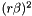- see below for details.
Returns
Correction factor to coulomb force - see below for details.

This routine is meant to enable analytical evaluation of the direct-space PME electrostatic force to avoid tables.

The direct-space potential should be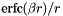, but there are some problems evaluating that:

First, the error function is difficult (read: expensive) to approxmiate accurately for intermediate to large arguments, and this happens already in ranges of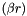that occur in simulations. Second, we now try to avoid calculating potentials in Gromacs but use forces directly.

We can simply things slight by noting that the PME part is really a correction to the normal Coulomb force since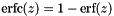, i.e.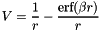The first term we already have from the inverse square root, so that we can leave out of this routine.

For pme tolerances of 1e-3 to 1e-8 and cutoffs of 0.5nm to 1.8nm, the argument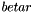will be in the range 0.15 to ~4, which is the range used for the minimax fit. Use your favorite plotting program to realize how well-behaved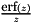is in this range!

We approximate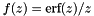with a rational minimax polynomial. However, it turns out it is more efficient to approximate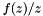and then only use even powers. This is another minor optimization, since we actually want, because it is going to be multiplied by the vector between the two atoms to get the vectorial force. The fastest flops are the ones we can avoid calculating!

So, here's how it should be used:

1. Calculate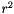.
2. Multiply by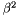, so you get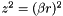.
3. Evaluate this routine with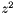as the argument.
4. The return value is the expression: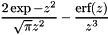5. Multiply the entire expression by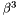. This will get you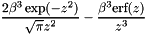or, switching back to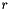(since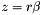):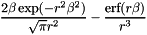With a bit of math exercise you should be able to confirm that this is exactly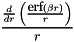6. Add the result to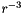, multiply by the product of the charges, and you have your force (divided by). A final multiplication with the vector connecting the two particles and you have your vectorial force to add to the particles.

This approximation achieves an error slightly lower than 1e-6 in single precision and 1e-11 in double precision for arguments smaller than 16 (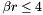); when added to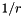the error will be insignificant. For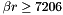the return value can be inf or NaN.

 static SimdDouble gmx_simdcall gmx::pmeForceCorrection ( SimdDouble z2 )
inlinestatic

Calculate the force correction due to PME analytically in SIMD double.

Parameters
 z2 This should be the value, where r is your interaction distance and beta the ewald splitting parameters.
Returns
Correction factor to coulomb force.

This routine is meant to enable analytical evaluation of the direct-space PME electrostatic force to avoid tables. For details, see the single precision function.

 static SimdDouble gmx_simdcall gmx::pmeForceCorrectionSingleAccuracy ( SimdDouble z2 )
static

Analytical PME force correction, double SIMD data, single accuracy.

Parameters
 z2- see below for details.
Returns
Correction factor to coulomb force - see below for details.

This routine is meant to enable analytical evaluation of the direct-space PME electrostatic force to avoid tables.

The direct-space potential should be, but there are some problems evaluating that:

First, the error function is difficult (read: expensive) to approxmiate accurately for intermediate to large arguments, and this happens already in ranges ofthat occur in simulations. Second, we now try to avoid calculating potentials in Gromacs but use forces directly.

We can simply things slight by noting that the PME part is really a correction to the normal Coulomb force since, i.e.The first term we already have from the inverse square root, so that we can leave out of this routine.

For pme tolerances of 1e-3 to 1e-8 and cutoffs of 0.5nm to 1.8nm, the argumentwill be in the range 0.15 to ~4. Use your favorite plotting program to realize how well-behavedis in this range!

We approximatewith a rational minimax polynomial. However, it turns out it is more efficient to approximateand then only use even powers. This is another minor optimization, since we actually want, because it is going to be multiplied by the vector between the two atoms to get the vectorial force. The fastest flops are the ones we can avoid calculating!

So, here's how it should be used:

1. Calculate.
2. Multiply by, so you get.
3. Evaluate this routine withas the argument.
4. The return value is the expression:5. Multiply the entire expression by. This will get youor, switching back to(since):With a bit of math exercise you should be able to confirm that this is exactly6. Add the result to, multiply by the product of the charges, and you have your force (divided by). A final multiplication with the vector connecting the two particles and you have your vectorial force to add to the particles.

This approximation achieves an accuracy slightly lower than 1e-6; when added tothe error will be insignificant.

 static SimdFloat gmx_simdcall gmx::pmeForceCorrectionSingleAccuracy ( SimdFloat z2 )
inlinestatic

SIMD Analytic PME force correction, only targeting single accuracy.

Parameters
 z2- see default single precision version for details.
Returns
Correction factor to coulomb force.
 static SimdFloat gmx_simdcall gmx::pmePotentialCorrection ( SimdFloat z2 )
inlinestatic

Calculate the potential correction due to PME analytically in SIMD float.

Parameters
 z2- see below for details.
Returns
Correction factor to coulomb potential - see below for details.

See pmeForceCorrection for details about the approximation.

This routine calculates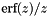, although you should provideas the input argument.

Here's how it should be used:

1. Calculate.
2. Multiply by, so you get.
3. Evaluate this routine with z^2 as the argument.
4. The return value is the expression: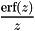5. Multiply the entire expression by beta and switching back to(since):6. Subtract the result from, multiply by the product of the charges, and you have your potential.

This approximation achieves an error slightly lower than 1e-6 in single precision and 4e-11 in double precision for arguments smaller than 16 (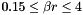); for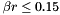the error can be twice as high; when added tothe error will be insignificant. For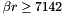the return value can be inf or NaN.

 static SimdDouble gmx_simdcall gmx::pmePotentialCorrection ( SimdDouble z2 )
inlinestatic

Calculate the potential correction due to PME analytically in SIMD double.

Parameters
 z2 This should be the value, where r is your interaction distance and beta the ewald splitting parameters.
Returns
Correction factor to coulomb force.

This routine is meant to enable analytical evaluation of the direct-space PME electrostatic potential to avoid tables. For details, see the single precision function.

 static SimdDouble gmx_simdcall gmx::pmePotentialCorrectionSingleAccuracy ( SimdDouble z2 )
static

Analytical PME potential correction, double SIMD data, single accuracy.

Parameters
 z2- see below for details.
Returns
Correction factor to coulomb potential - see below for details.

This routine calculates, although you should provideas the input argument.

Here's how it should be used:

1. Calculate.
2. Multiply by, so you get.
3. Evaluate this routine with z^2 as the argument.
4. The return value is the expression:5. Multiply the entire expression by beta and switching back to(since):6. Subtract the result from, multiply by the product of the charges, and you have your potential.

This approximation achieves an accuracy slightly lower than 1e-6; when added tothe error will be insignificant.

 static SimdFloat gmx_simdcall gmx::pmePotentialCorrectionSingleAccuracy ( SimdFloat z2 )
inlinestatic

SIMD Analytic PME potential correction, only targeting single accuracy.

Parameters
 z2- see default single precision version for details.
Returns
Correction factor to coulomb force.
 static SimdFloat gmx_simdcall gmx::rcpIter ( SimdFloat lu, SimdFloat x )
inlinestatic

Perform one Newton-Raphson iteration to improve 1/x for SIMD float.

This is a low-level routine that should only be used by SIMD math routine that evaluates the reciprocal.

Parameters
 lu Approximation of 1/x, typically obtained from lookup. x The reference (starting) value x for which we want 1/x.
Returns
An improved approximation with roughly twice as many bits of accuracy.
 static SimdDouble gmx_simdcall gmx::rcpIter ( SimdDouble lu, SimdDouble x )
inlinestatic

Perform one Newton-Raphson iteration to improve 1/x for SIMD double.

This is a low-level routine that should only be used by SIMD math routine that evaluates the reciprocal.

Parameters
 lu Approximation of 1/x, typically obtained from lookup. x The reference (starting) value x for which we want 1/x.
Returns
An improved approximation with roughly twice as many bits of accuracy.
 static float gmx_simdcall gmx::reduce ( Simd4Float a )
inlinestatic

Return sum of all elements in SIMD4 float variable.

Parameters
 a SIMD4 variable to reduce/sum.
Returns
The sum of all elements in the argument variable.
 static double gmx_simdcall gmx::reduce ( Simd4Double a )
inlinestatic

Return sum of all elements in SIMD4 double variable.

Parameters
 a SIMD4 variable to reduce/sum.
Returns
The sum of all elements in the argument variable.
 static double gmx_simdcall gmx::reduceIncr4ReturnSum ( double * m, SimdDouble v0, SimdDouble v1, SimdDouble v2, SimdDouble v3 )
inlinestatic

Reduce each of four SIMD doubles, add those values to four consecutive doubles in memory, return sum.

Parameters
 m Pointer to memory where four doubles should be incremented v0 SIMD variable whose sum should be added to m v1 SIMD variable whose sum should be added to m v2 SIMD variable whose sum should be added to m v3 SIMD variable whose sum should be added to m
Returns
Sum of all elements in the four SIMD variables.

The pointer m must be aligned to the smaller of four elements and the floating-point SIMD width.

Note
This is a special routine intended for the Gromacs nonbonded kernels. It is used in the epilogue of the outer loop, where the variables will contain unrolled forces for one outer-loop-particle each, corresponding to a single coordinate (i.e, say, four x-coordinate force variables). These should be summed and added to the force array in memory. Since we always work with contiguous SIMD-layout , we can use efficient aligned loads/stores. When calculating the virial, we also need the total sum of all forces for each coordinate. This is provided as the return value. For routines that do not need these, this extra code will be optimized away completely if you just ignore the return value (Checked with gcc-4.9.1 and clang-3.6 for AVX).
 static float gmx_simdcall gmx::reduceIncr4ReturnSum ( float * m, SimdFloat v0, SimdFloat v1, SimdFloat v2, SimdFloat v3 )
inlinestatic

Reduce each of four SIMD floats, add those values to four consecutive floats in memory, return sum.

Parameters
 m Pointer to memory where four floats should be incremented v0 SIMD variable whose sum should be added to m v1 SIMD variable whose sum should be added to m v2 SIMD variable whose sum should be added to m v3 SIMD variable whose sum should be added to m
Returns
Sum of all elements in the four SIMD variables.

The pointer m must be aligned to the smaller of four elements and the floating-point SIMD width.

Note
This is a special routine intended for the Gromacs nonbonded kernels. It is used in the epilogue of the outer loop, where the variables will contain unrolled forces for one outer-loop-particle each, corresponding to a single coordinate (i.e, say, four x-coordinate force variables). These should be summed and added to the force array in memory. Since we always work with contiguous SIMD-layout , we can use efficient aligned loads/stores. When calculating the virial, we also need the total sum of all forces for each coordinate. This is provided as the return value. For routines that do not need these, this extra code will be optimized away completely if you just ignore the return value (Checked with gcc-4.9.1 and clang-3.6 for AVX).
 static Simd4Float gmx_simdcall gmx::round ( Simd4Float a )
inlinestatic

SIMD4 Round to nearest integer value (in floating-point format).

Parameters
 a Any floating-point value
Returns
The nearest integer, represented in floating-point format.
 static Simd4Double gmx_simdcall gmx::round ( Simd4Double a )
inlinestatic

SIMD4 Round to nearest integer value (in floating-point format).

Parameters
 a Any floating-point value
Returns
The nearest integer, represented in floating-point format.
 static Simd4Double gmx_simdcall gmx::rsqrt ( Simd4Double x )
inlinestatic

SIMD4 1.0/sqrt(x) lookup.

This is a low-level instruction that should only be called from routines implementing the inverse square root in simd_math.h.

Parameters
 x Argument, x>0
Returns
Approximation of 1/sqrt(x), accuracy is GMX_SIMD_RSQRT_BITS.
 static Simd4Float gmx_simdcall gmx::rsqrt ( Simd4Float x )
inlinestatic

SIMD4 1.0/sqrt(x) lookup.

This is a low-level instruction that should only be called from routines implementing the inverse square root in simd_math.h.

Parameters
 x Argument, x>0
Returns
Approximation of 1/sqrt(x), accuracy is GMX_SIMD_RSQRT_BITS.
 static SimdFloat gmx_simdcall gmx::rsqrtIter ( SimdFloat lu, SimdFloat x )
inlinestatic

Perform one Newton-Raphson iteration to improve 1/sqrt(x) for SIMD float.

This is a low-level routine that should only be used by SIMD math routine that evaluates the inverse square root.

Parameters
 lu Approximation of 1/sqrt(x), typically obtained from lookup. x The reference (starting) value x for which we want 1/sqrt(x).
Returns
An improved approximation with roughly twice as many bits of accuracy.
 static SimdDouble gmx_simdcall gmx::rsqrtIter ( SimdDouble lu, SimdDouble x )
inlinestatic

Perform one Newton-Raphson iteration to improve 1/sqrt(x) for SIMD double.

This is a low-level routine that should only be used by SIMD math routine that evaluates the inverse square root.

Parameters
 lu Approximation of 1/sqrt(x), typically obtained from lookup. x The reference (starting) value x for which we want 1/sqrt(x).
Returns
An improved approximation with roughly twice as many bits of accuracy.
 static Simd4Float gmx_simdcall gmx::rsqrtIter ( Simd4Float lu, Simd4Float x )
inlinestatic

Perform one Newton-Raphson iteration to improve 1/sqrt(x) for SIMD4 float.

This is a low-level routine that should only be used by SIMD math routine that evaluates the inverse square root.

Parameters
 lu Approximation of 1/sqrt(x), typically obtained from lookup. x The reference (starting) value x for which we want 1/sqrt(x).
Returns
An improved approximation with roughly twice as many bits of accuracy.
 static Simd4Double gmx_simdcall gmx::rsqrtIter ( Simd4Double lu, Simd4Double x )
inlinestatic

Perform one Newton-Raphson iteration to improve 1/sqrt(x) for SIMD4 double.

This is a low-level routine that should only be used by SIMD math routine that evaluates the inverse square root.

Parameters
 lu Approximation of 1/sqrt(x), typically obtained from lookup. x The reference (starting) value x for which we want 1/sqrt(x).
Returns
An improved approximation with roughly twice as many bits of accuracy.
 static Simd4Float gmx_simdcall gmx::selectByMask ( Simd4Float a, Simd4FBool mask )
inlinestatic

Select from single precision SIMD4 variable where boolean is true.

Parameters
 a Floating-point variable to select from mask Boolean selector
Returns
For each element, a is selected for true, 0 for false.
 static Simd4Double gmx_simdcall gmx::selectByMask ( Simd4Double a, Simd4DBool mask )
inlinestatic

Select from single precision SIMD4 variable where boolean is true.

Parameters
 a Floating-point variable to select from mask Boolean selector
Returns
For each element, a is selected for true, 0 for false.
 static Simd4Float gmx_simdcall gmx::selectByNotMask ( Simd4Float a, Simd4FBool mask )
inlinestatic

Select from single precision SIMD4 variable where boolean is false.

Parameters
 a Floating-point variable to select from mask Boolean selector
Returns
For each element, a is selected for false, 0 for true (sic).
 static Simd4Double gmx_simdcall gmx::selectByNotMask ( Simd4Double a, Simd4DBool mask )
inlinestatic

Select from single precision SIMD4 variable where boolean is false.

Parameters
 a Floating-point variable to select from mask Boolean selector
Returns
For each element, a is selected for false, 0 for true (sic).
 static Simd4Double gmx_simdcall gmx::simd4SetZeroD ( )
inlinestatic

Set all SIMD4 double elements to 0.

You should typically just call gmx::setZero(), which uses proxy objects internally to handle all types rather than adding the suffix used here.

Returns
SIMD4 0.0
 static Simd4Float gmx_simdcall gmx::simd4SetZeroF ( )
inlinestatic

Set all SIMD4 float elements to 0.

You should typically just call gmx::setZero(), which uses proxy objects internally to handle all types rather than adding the suffix used here.

Returns
SIMD4 0.0
 static SimdFloat gmx_simdcall gmx::sin ( SimdFloat x )
inlinestatic

SIMD float sin(x).

Parameters
 x The argument to evaluate sin for
Returns
Sin(x)
Attention
Do NOT call both sin & cos if you need both results, since each of them will then call sincos and waste a factor 2 in performance.
 static SimdDouble gmx_simdcall gmx::sin ( SimdDouble x )
inlinestatic

SIMD double sin(x).

Parameters
 x The argument to evaluate sin for
Returns
Sin(x)
Attention
Do NOT call both sin & cos if you need both results, since each of them will then call sincos and waste a factor 2 in performance.
 static void gmx_simdcall gmx::sincos ( SimdFloat x, SimdFloat * sinval, SimdFloat * cosval )
inlinestatic

SIMD float sin & cos.

Parameters
 x The argument to evaluate sin/cos for [out] sinval Sin(x) [out] cosval Cos(x)

This version achieves close to machine precision, but for very large magnitudes of the argument we inherently begin to lose accuracy due to the argument reduction, despite using extended precision arithmetics internally.

 static void gmx_simdcall gmx::sincos ( SimdDouble x, SimdDouble * sinval, SimdDouble * cosval )
inlinestatic

SIMD double sin & cos.

Parameters
 x The argument to evaluate sin/cos for [out] sinval Sin(x) [out] cosval Cos(x)

This version achieves close to machine precision, but for very large magnitudes of the argument we inherently begin to lose accuracy due to the argument reduction, despite using extended precision arithmetics internally.

 static void gmx_simdcall gmx::sinCosSingleAccuracy ( SimdDouble x, SimdDouble * sinval, SimdDouble * cosval )
inlinestatic

SIMD sin & cos. Double precision SIMD data, single accuracy.

Parameters
 x The argument to evaluate sin/cos for [out] sinval Sin(x) [out] cosval Cos(x)
 static void gmx_simdcall gmx::sinCosSingleAccuracy ( SimdFloat x, SimdFloat * sinval, SimdFloat * cosval )
inlinestatic

SIMD float sin & cos, only targeting single accuracy.

Parameters
 x The argument to evaluate sin/cos for [out] sinval Sin(x) [out] cosval Cos(x)
 static SimdDouble gmx_simdcall gmx::sinSingleAccuracy ( SimdDouble x )
inlinestatic

SIMD sin(x). Double precision SIMD data, single accuracy.

Parameters
 x The argument to evaluate sin for
Returns
Sin(x)
Attention
Do NOT call both sin & cos if you need both results, since each of them will then call sincos and waste a factor 2 in performance.
 static SimdFloat gmx_simdcall gmx::sinSingleAccuracy ( SimdFloat x )
inlinestatic

SIMD float sin(x), only targeting single accuracy.

Parameters
 x The argument to evaluate sin for
Returns
Sin(x)
Attention
Do NOT call both sin & cos if you need both results, since each of them will then call sincos and waste a factor 2 in performance.
 static SimdFloat gmx_simdcall gmx::sqrt ( SimdFloat x )
inlinestatic

Calculate sqrt(x) correctly for SIMD floats, including argument 0.0.

Parameters
 x Argument that must be >=0.
Returns
sqrt(x). If x=0, the result will correctly be set to 0. The result is undefined if the input value is negative.
 static SimdDouble gmx_simdcall gmx::sqrt ( SimdDouble x )
inlinestatic

Calculate sqrt(x) correctly for SIMD doubles, including argument 0.0.

Parameters
 x Argument that must be >=0.
Returns
sqrt(x). If x=0, the result will correctly be set to 0. if x>0 && x<float_min, the result will incorrectly be set to 0. The result is undefined if the input value is negative.
 static SimdDouble gmx_simdcall gmx::sqrtSingleAccuracy ( SimdDouble x )
inlinestatic

Calculate sqrt(x) (correct for 0.0) for SIMD double, single accuracy.

Parameters
 x Argument that must be >=0.
Returns
sqrt(x). If x<float_min, the result will correctly be set to 0. The result is undefined if the input value is negative.
 static SimdFloat gmx_simdcall gmx::sqrtSingleAccuracy ( SimdFloat x )
inlinestatic

Calculate sqrt(x) for SIMD float, only targeting single accuracy.

Parameters
 x Argument that must be >=0.
Returns
sqrt(x). If x=0, the result will correctly be set to 0. The result is undefined if the input value is negative.
 static void gmx_simdcall gmx::store4 ( double * m, Simd4Double a )
inlinestatic

Store the contents of SIMD4 double to aligned memory m.

Parameters
 [out] m Pointer to memory, aligned to 4 elements. a SIMD4 variable to store
 static void gmx_simdcall gmx::store4 ( float * m, Simd4Float a )
inlinestatic

Store the contents of SIMD4 float to aligned memory m.

Parameters
 [out] m Pointer to memory, aligned to 4 elements. a SIMD4 variable to store
 static void gmx_simdcall gmx::store4U ( float * m, Simd4Float a )
inlinestatic

Store SIMD4 float to unaligned memory.

Available if GMX_SIMD_HAVE_STOREU is 1.

Parameters
 [out] m Pointer to memory, no alignment requirement. a SIMD4 variable to store.
 static void gmx_simdcall gmx::store4U ( double * m, Simd4Double a )
inlinestatic

Store SIMD4 double to unaligned memory.

Available if GMX_SIMD_HAVE_STOREU is 1.

Parameters
 [out] m Pointer to memory, no alignment requirement. a SIMD4 variable to store.
 static SimdFloat gmx_simdcall gmx::tan ( SimdFloat x )
inlinestatic

SIMD float tan(x).

Parameters
 x The argument to evaluate tan for
Returns
Tan(x)
 static SimdDouble gmx_simdcall gmx::tan ( SimdDouble x )
inlinestatic

SIMD double tan(x).

Parameters
 x The argument to evaluate tan for
Returns
Tan(x)
 static SimdDouble gmx_simdcall gmx::tanSingleAccuracy ( SimdDouble x )
inlinestatic

SIMD tan(x). Double precision SIMD data, single accuracy.

Parameters
 x The argument to evaluate tan for
Returns
Tan(x)
 static SimdFloat gmx_simdcall gmx::tanSingleAccuracy ( SimdFloat x )
inlinestatic

SIMD float tan(x), only targeting single accuracy.

Parameters
 x The argument to evaluate tan for
Returns
Tan(x)
 static void gmx_simdcall gmx::transpose ( Simd4Float * v0, Simd4Float * v1, Simd4Float * v2, Simd4Float * v3 )
inlinestatic

SIMD4 float transpose.

Parameters
 [in,out] v0 Row 0 on input, column 0 on output [in,out] v1 Row 1 on input, column 1 on output [in,out] v2 Row 2 on input, column 2 on output [in,out] v3 Row 3 on input, column 3 on output
 static void gmx_simdcall gmx::transpose ( Simd4Double * v0, Simd4Double * v1, Simd4Double * v2, Simd4Double * v3 )
inlinestatic

SIMD4 double transpose.

Parameters
 [in,out] v0 Row 0 on input, column 0 on output [in,out] v1 Row 1 on input, column 1 on output [in,out] v2 Row 2 on input, column 2 on output [in,out] v3 Row 3 on input, column 3 on output
template<int align>
 static void gmx_simdcall gmx::transposeScatterDecrU ( double * base, const std::int32_t offset[], SimdDouble v0, SimdDouble v1, SimdDouble v2 )
inlinestatic

Transpose and subtract 3 SIMD doubles to 3 consecutive addresses at GMX_SIMD_DOUBLE_WIDTH offsets.

Template Parameters
 align Alignment of the memory to which we write, i.e. distance (measured in elements, not bytes) between index points. When this is identical to the number of SIMD variables (i.e., 3 for this routine) the output data is packed without padding in memory. See the SIMD parameters for exactly what memory positions are decremented.
Parameters
 [out] base Pointer to start of memory. offset Aligned array with offsets to the start of each triplet. v0 1st component, subtracted from base[align*offset[i]] v1 2nd component, subtracted from base[align*offset[i]+1] v2 3rd component, subtracted from base[align*offset[i]+2]

This function can work with both aligned (better performance) and unaligned memory. When the align parameter is not a power-of-two (align==3 would be normal for packed atomic coordinates) the memory obviously cannot be aligned, and we account for this. However, in the case where align is a power-of-two, we assume the base pointer also has the same alignment, which will enable many platforms to use faster aligned memory load/store operations. An easy way to think of this is that each triplet of data in memory must be aligned to the align parameter you specify when it's a power-of-two.

The offset memory must always be aligned to GMX_SIMD_FINT32_WIDTH, since this enables us to use SIMD loads and gather operations on platforms that support it.

Note
You should NOT scale offsets before calling this routine; it is done internally by using the alignment template parameter instead.
This routine uses a normal array for the offsets, since we typically load the data from memory. On the architectures we have tested this is faster even when a SIMD integer datatype is present.
To improve performance, this function might use full-SIMD-width unaligned load/store, and subtract 0.0 from the extra elements. This means you need to ensure the memory is padded at the end, so we always can load GMX_SIMD_REAL_WIDTH elements starting at the last offset. If you use the Gromacs aligned memory allocation routines this will always be the case.
template<int align>
 static void gmx_simdcall gmx::transposeScatterDecrU ( float * base, const std::int32_t offset[], SimdFloat v0, SimdFloat v1, SimdFloat v2 )
inlinestatic

Transpose and subtract 3 SIMD floats to 3 consecutive addresses at GMX_SIMD_FLOAT_WIDTH offsets.

Template Parameters
 align Alignment of the memory to which we write, i.e. distance (measured in elements, not bytes) between index points. When this is identical to the number of SIMD variables (i.e., 3 for this routine) the output data is packed without padding in memory. See the SIMD parameters for exactly what memory positions are decremented.
Parameters
 [out] base Pointer to start of memory. offset Aligned array with offsets to the start of each triplet. v0 1st component, subtracted from base[align*offset[i]] v1 2nd component, subtracted from base[align*offset[i]+1] v2 3rd component, subtracted from base[align*offset[i]+2]

This function can work with both aligned (better performance) and unaligned memory. When the align parameter is not a power-of-two (align==3 would be normal for packed atomic coordinates) the memory obviously cannot be aligned, and we account for this. However, in the case where align is a power-of-two, we assume the base pointer also has the same alignment, which will enable many platforms to use faster aligned memory load/store operations. An easy way to think of this is that each triplet of data in memory must be aligned to the align parameter you specify when it's a power-of-two.

The offset memory must always be aligned to GMX_SIMD_FINT32_WIDTH, since this enables us to use SIMD loads and gather operations on platforms that support it.

Note
You should NOT scale offsets before calling this routine; it is done internally by using the alignment template parameter instead.
This routine uses a normal array for the offsets, since we typically load the data from memory. On the architectures we have tested this is faster even when a SIMD integer datatype is present.
To improve performance, this function might use full-SIMD-width unaligned load/store, and subtract 0.0 from the extra elements. This means you need to ensure the memory is padded at the end, so we always can load GMX_SIMD_REAL_WIDTH elements starting at the last offset. If you use the Gromacs aligned memory allocation routines this will always be the case.
template<int align>
 static void gmx_simdcall gmx::transposeScatterIncrU ( double * base, const std::int32_t offset[], SimdDouble v0, SimdDouble v1, SimdDouble v2 )
inlinestatic

Transpose and add 3 SIMD doubles to 3 consecutive addresses at GMX_SIMD_DOUBLE_WIDTH offsets.

Template Parameters
 align Alignment of the memory to which we write, i.e. distance (measured in elements, not bytes) between index points. When this is identical to the number of SIMD variables (i.e., 3 for this routine) the output data is packed without padding in memory. See the SIMD parameters for exactly what memory positions are incremented.
Parameters
 [out] base Pointer to the start of the memory area offset Aligned array with offsets to the start of each triplet. v0 1st component of triplets, added to base[align*offset[i]]. v1 2nd component of triplets, added to base[align*offset[i] + 1]. v2 3rd component of triplets, added to base[align*offset[i] + 2].

This function can work with both aligned (better performance) and unaligned memory. When the align parameter is not a power-of-two (align==3 would be normal for packed atomic coordinates) the memory obviously cannot be aligned, and we account for this. However, in the case where align is a power-of-two, we assume the base pointer also has the same alignment, which will enable many platforms to use faster aligned memory load/store operations. An easy way to think of this is that each triplet of data in memory must be aligned to the align parameter you specify when it's a power-of-two.

The offset memory must always be aligned to GMX_SIMD_FINT32_WIDTH, since this enables us to use SIMD loads and gather operations on platforms that support it.

Note
You should NOT scale offsets before calling this routine; it is done internally by using the alignment template parameter instead.
This routine uses a normal array for the offsets, since we typically load the data from memory. On the architectures we have tested this is faster even when a SIMD integer datatype is present.
To improve performance, this function might use full-SIMD-width unaligned load/store, and add 0.0 to the extra elements. This means you need to ensure the memory is padded at the end, so we always can load GMX_SIMD_REAL_WIDTH elements starting at the last offset. If you use the Gromacs aligned memory allocation routines this will always be the case.
template<int align>
 static void gmx_simdcall gmx::transposeScatterIncrU ( float * base, const std::int32_t offset[], SimdFloat v0, SimdFloat v1, SimdFloat v2 )
inlinestatic

Transpose and add 3 SIMD floats to 3 consecutive addresses at GMX_SIMD_FLOAT_WIDTH offsets.

Template Parameters
 align Alignment of the memory to which we write, i.e. distance (measured in elements, not bytes) between index points. When this is identical to the number of SIMD variables (i.e., 3 for this routine) the output data is packed without padding in memory. See the SIMD parameters for exactly what memory positions are incremented.
Parameters
 [out] base Pointer to the start of the memory area offset Aligned array with offsets to the start of each triplet. v0 1st component of triplets, added to base[align*offset[i]]. v1 2nd component of triplets, added to base[align*offset[i] + 1]. v2 3rd component of triplets, added to base[align*offset[i] + 2].

This function can work with both aligned (better performance) and unaligned memory. When the align parameter is not a power-of-two (align==3 would be normal for packed atomic coordinates) the memory obviously cannot be aligned, and we account for this. However, in the case where align is a power-of-two, we assume the base pointer also has the same alignment, which will enable many platforms to use faster aligned memory load/store operations. An easy way to think of this is that each triplet of data in memory must be aligned to the align parameter you specify when it's a power-of-two.

The offset memory must always be aligned to GMX_SIMD_FINT32_WIDTH, since this enables us to use SIMD loads and gather operations on platforms that support it.

Note
You should NOT scale offsets before calling this routine; it is done internally by using the alignment template parameter instead.
This routine uses a normal array for the offsets, since we typically load the data from memory. On the architectures we have tested this is faster even when a SIMD integer datatype is present.
To improve performance, this function might use full-SIMD-width unaligned load/store, and add 0.0 to the extra elements. This means you need to ensure the memory is padded at the end, so we always can load GMX_SIMD_REAL_WIDTH elements starting at the last offset. If you use the Gromacs aligned memory allocation routines this will always be the case.
template<int align>
 static void gmx_simdcall gmx::transposeScatterStoreU ( double * base, const std::int32_t offset[], SimdDouble v0, SimdDouble v1, SimdDouble v2 )
inlinestatic

Transpose and store 3 SIMD doubles to 3 consecutive addresses at GMX_SIMD_DOUBLE_WIDTH offsets.

Template Parameters
 align Alignment of the memory to which we write, i.e. distance (measured in elements, not bytes) between index points. When this is identical to the number of SIMD variables (i.e., 3 for this routine) the output data is packed without padding in memory. See the SIMD parameters for exactly what memory positions are written.
Parameters
 [out] base Pointer to the start of the memory area offset Aligned array with offsets to the start of each triplet. v0 1st component of triplets, written to base[align*offset[i]]. v1 2nd component of triplets, written to base[align*offset[i] + 1]. v2 3rd component of triplets, written to base[align*offset[i] + 2].

This function can work with both aligned (better performance) and unaligned memory. When the align parameter is not a power-of-two (align==3 would be normal for packed atomic coordinates) the memory obviously cannot be aligned, and we account for this. However, in the case where align is a power-of-two, we assume the base pointer also has the same alignment, which will enable many platforms to use faster aligned memory store operations. An easy way to think of this is that each triplet of data in memory must be aligned to the align parameter you specify when it's a power-of-two.

The offset memory must always be aligned to GMX_SIMD_FINT32_WIDTH, since this enables us to use SIMD loads and gather operations on platforms that support it.

Note
You should NOT scale offsets before calling this routine; it is done internally by using the alignment template parameter instead.
This routine uses a normal array for the offsets, since we typically load the data from memory. On the architectures we have tested this is faster even when a SIMD integer datatype is present.
template<int align>
 static void gmx_simdcall gmx::transposeScatterStoreU ( float * base, const std::int32_t offset[], SimdFloat v0, SimdFloat v1, SimdFloat v2 )
inlinestatic

Transpose and store 3 SIMD floats to 3 consecutive addresses at GMX_SIMD_FLOAT_WIDTH offsets.

Template Parameters
 align Alignment of the memory to which we write, i.e. distance (measured in elements, not bytes) between index points. When this is identical to the number of SIMD variables (i.e., 3 for this routine) the output data is packed without padding in memory. See the SIMD parameters for exactly what memory positions are written.
Parameters
 [out] base Pointer to the start of the memory area offset Aligned array with offsets to the start of each triplet. v0 1st component of triplets, written to base[align*offset[i]]. v1 2nd component of triplets, written to base[align*offset[i] + 1]. v2 3rd component of triplets, written to base[align*offset[i] + 2].

This function can work with both aligned (better performance) and unaligned memory. When the align parameter is not a power-of-two (align==3 would be normal for packed atomic coordinates) the memory obviously cannot be aligned, and we account for this. However, in the case where align is a power-of-two, we assume the base pointer also has the same alignment, which will enable many platforms to use faster aligned memory store operations. An easy way to think of this is that each triplet of data in memory must be aligned to the align parameter you specify when it's a power-of-two.

The offset memory must always be aligned to GMX_SIMD_FINT32_WIDTH, since this enables us to use SIMD loads and gather operations on platforms that support it.

Note
You should NOT scale offsets before calling this routine; it is done internally by using the alignment template parameter instead.
This routine uses a normal array for the offsets, since we typically load the data from memory. On the architectures we have tested this is faster even when a SIMD integer datatype is present.
 static Simd4Float gmx_simdcall gmx::trunc ( Simd4Float a )
inlinestatic

Truncate SIMD4, i.e. round towards zero - common hardware instruction.

Parameters
 a Any floating-point value
Returns
Integer rounded towards zero, represented in floating-point format.
Note
This is truncation towards zero, not floor(). The reason for this is that truncation is virtually always present as a dedicated hardware instruction, but floor() frequently isn't.
 static Simd4Double gmx_simdcall gmx::trunc ( Simd4Double a )
inlinestatic

Truncate SIMD4, i.e. round towards zero - common hardware instruction.

Parameters
 a Any floating-point value
Returns
Integer rounded towards zero, represented in floating-point format.
Note
This is truncation towards zero, not floor(). The reason for this is that truncation is virtually always present as a dedicated hardware instruction, but floor() frequently isn't.

## Variable Documentation

 const int gmx::c_simdBestPairAlignmentDouble = 2
static

Best alignment to use for aligned pairs of double data.

The routines to load and transpose data will work with a wide range of alignments, but some might be faster than others, depending on the load instructions available in the hardware. This specifies the best alignment for each implementation when working with pairs of data.

To allow each architecture to use the most optimal form, we use a constant that code outside the SIMD module should use to store things properly. It must be at least 2. For example, a value of 2 means the two parameters A & B are stored as [A0 B0 A1 B1] while align-4 means [A0 B0 - - A1 B1 - -].

This alignment depends on the efficiency of partial-register load/store operations, and will depend on the architecture.

 const int gmx::c_simdBestPairAlignmentFloat = 2
static

Best alignment to use for aligned pairs of float data.

The routines to load and transpose data will work with a wide range of alignments, but some might be faster than others, depending on the load instructions available in the hardware. This specifies the best alignment for each implementation when working with pairs of data.

To allow each architecture to use the most optimal form, we use a constant that code outside the SIMD module should use to store things properly. It must be at least 2. For example, a value of 2 means the two parameters A & B are stored as [A0 B0 A1 B1] while align-4 means [A0 B0 - - A1 B1 - -].

This alignment depends on the efficiency of partial-register load/store operations, and will depend on the architecture.